Learn about smartphone sensors in the next Xcel masterclass with Tinkerbee CEO, Anupam! Learn about smartphone sensors in the next Xcel masterclass with Tinkerbee CEO, Anupam!

# Madhya Pradesh Board Question Paper for Class 10th Maths 2015 In PDF

## MP Board (MPBSE) Question Paper 2015 Class 10th Mathematics For English Medium with Solutions – Free Download

MP Board Question Paper 2015 Class 10th Maths with solutions are provided on this page by BYJU’S in downloadable pdf format and also in the text, so that the students prepare more efficiently for their final exams. Maths Question Paper 2015 Class 10 is also added here which assists in the exam preparation within the stipulated time. Students are able to access all the Madhya Pradesh board previous year maths question papers. Students gain more confidence by practising different types of questions from the previous year papers and get acquainted with the paper pattern that one can expect for the board exams.

### MPBSE Class 10th Maths Question Paper With Solutions 2015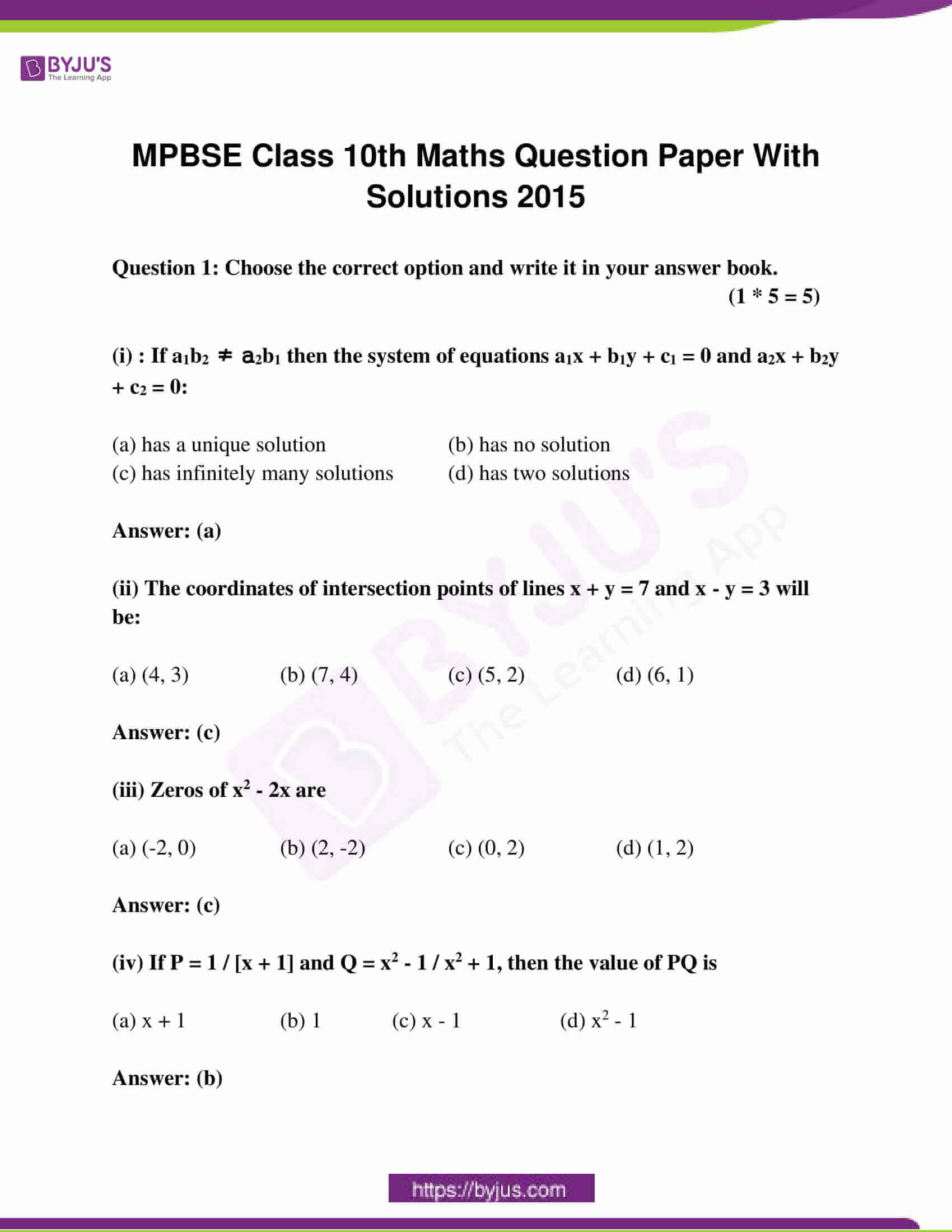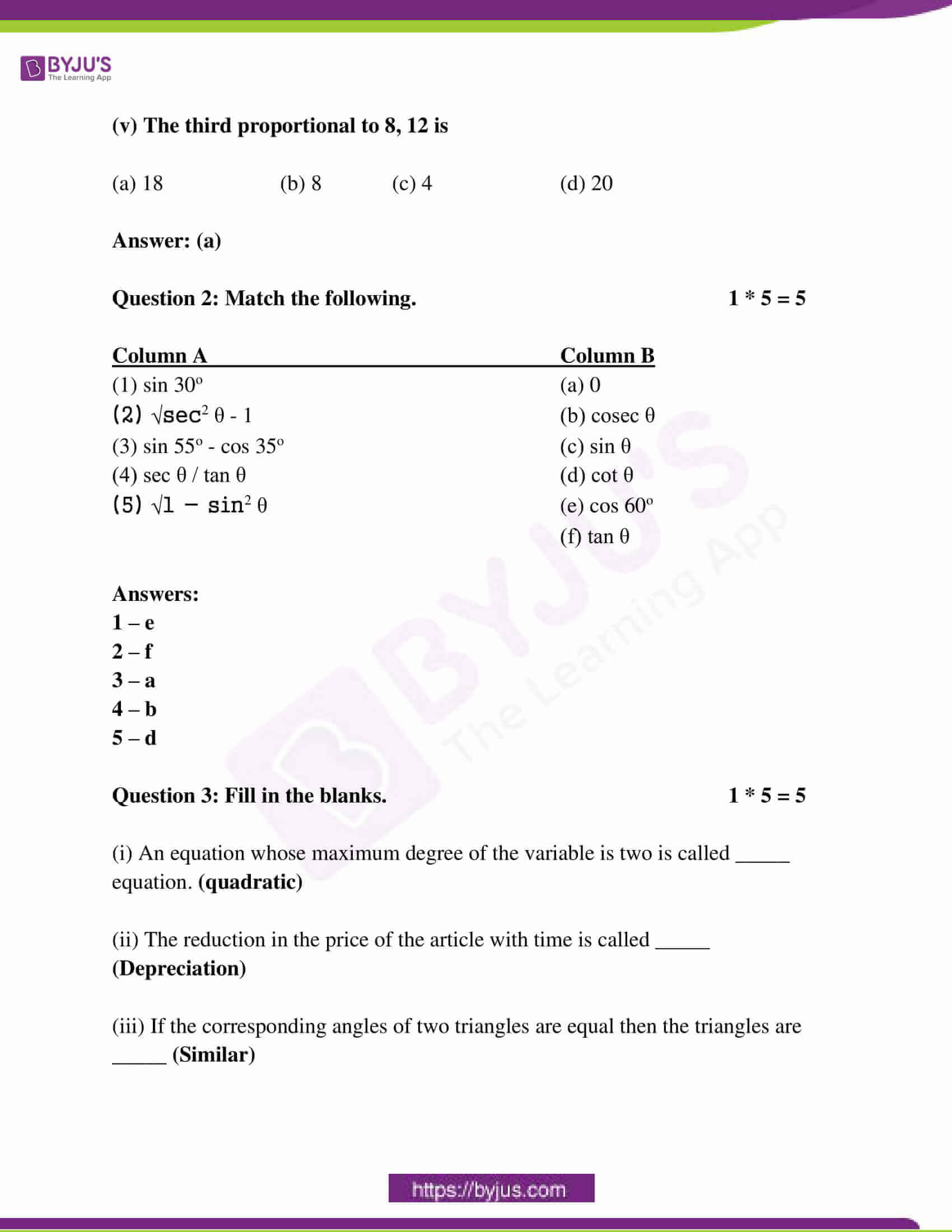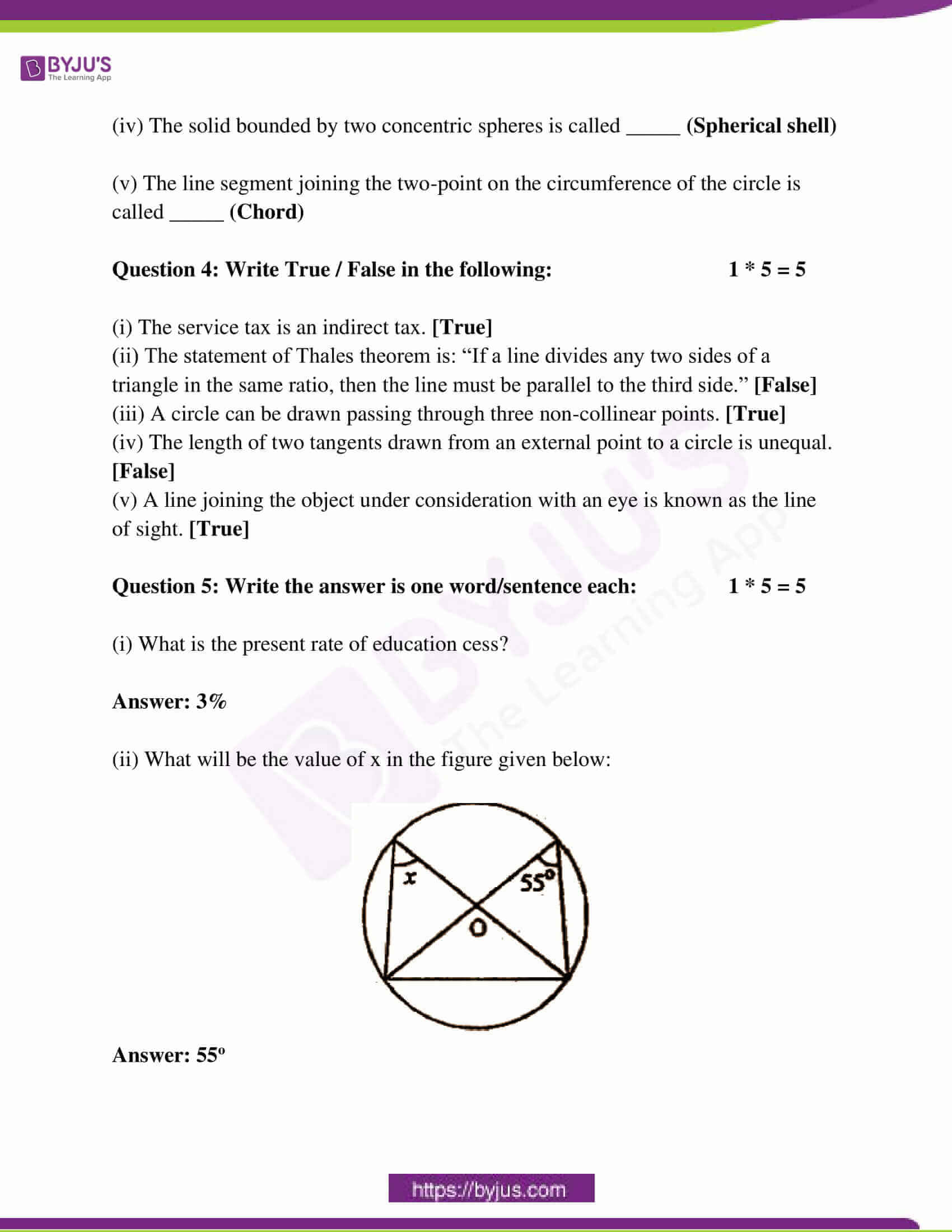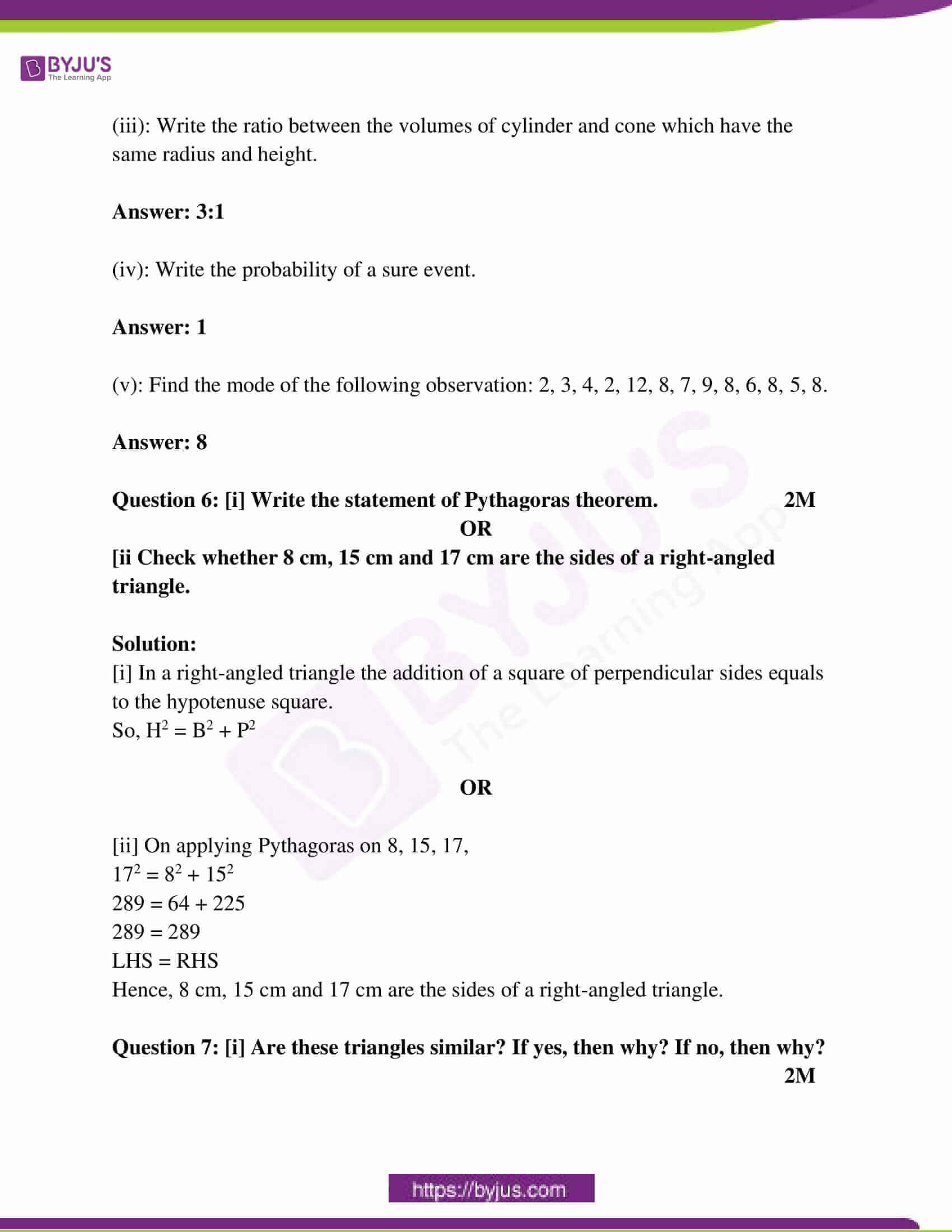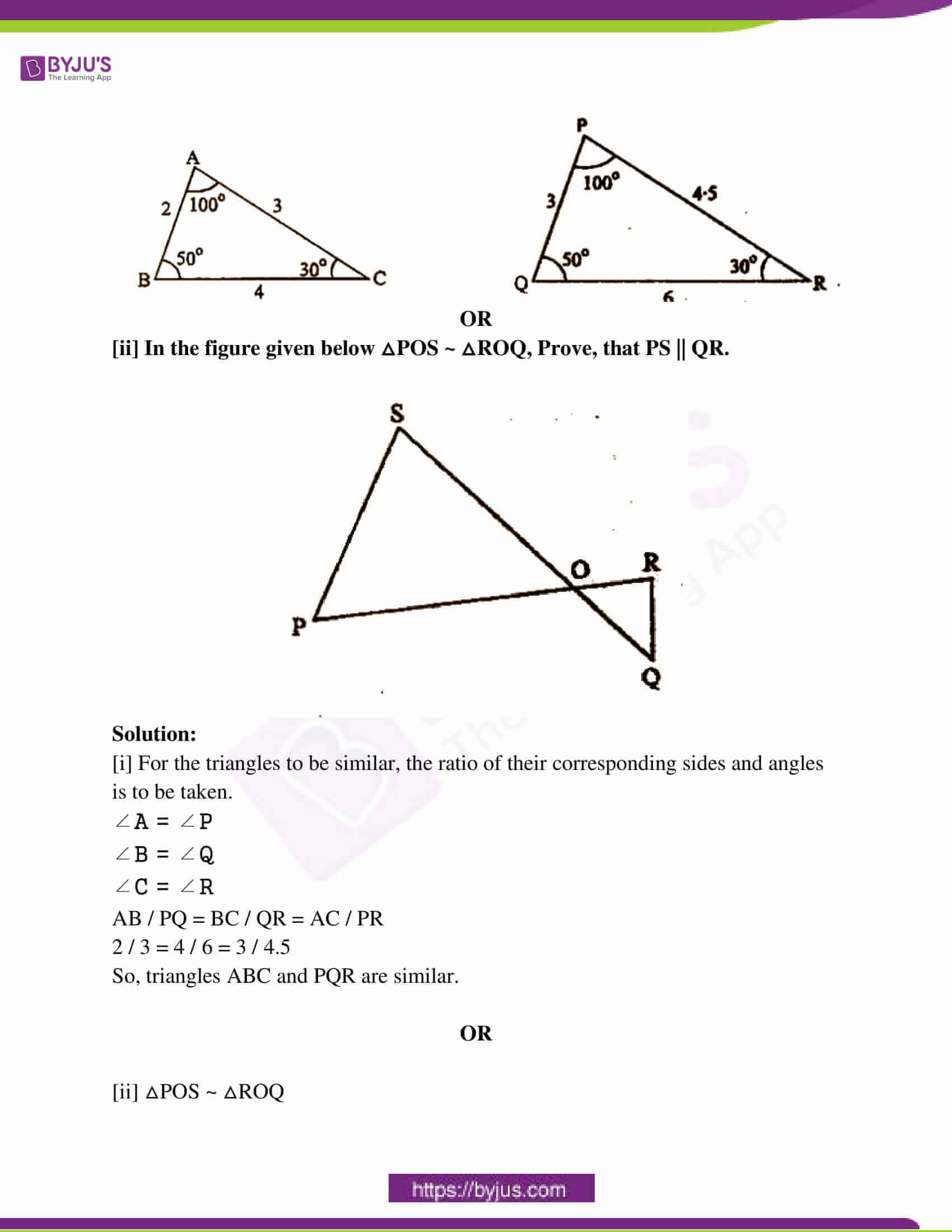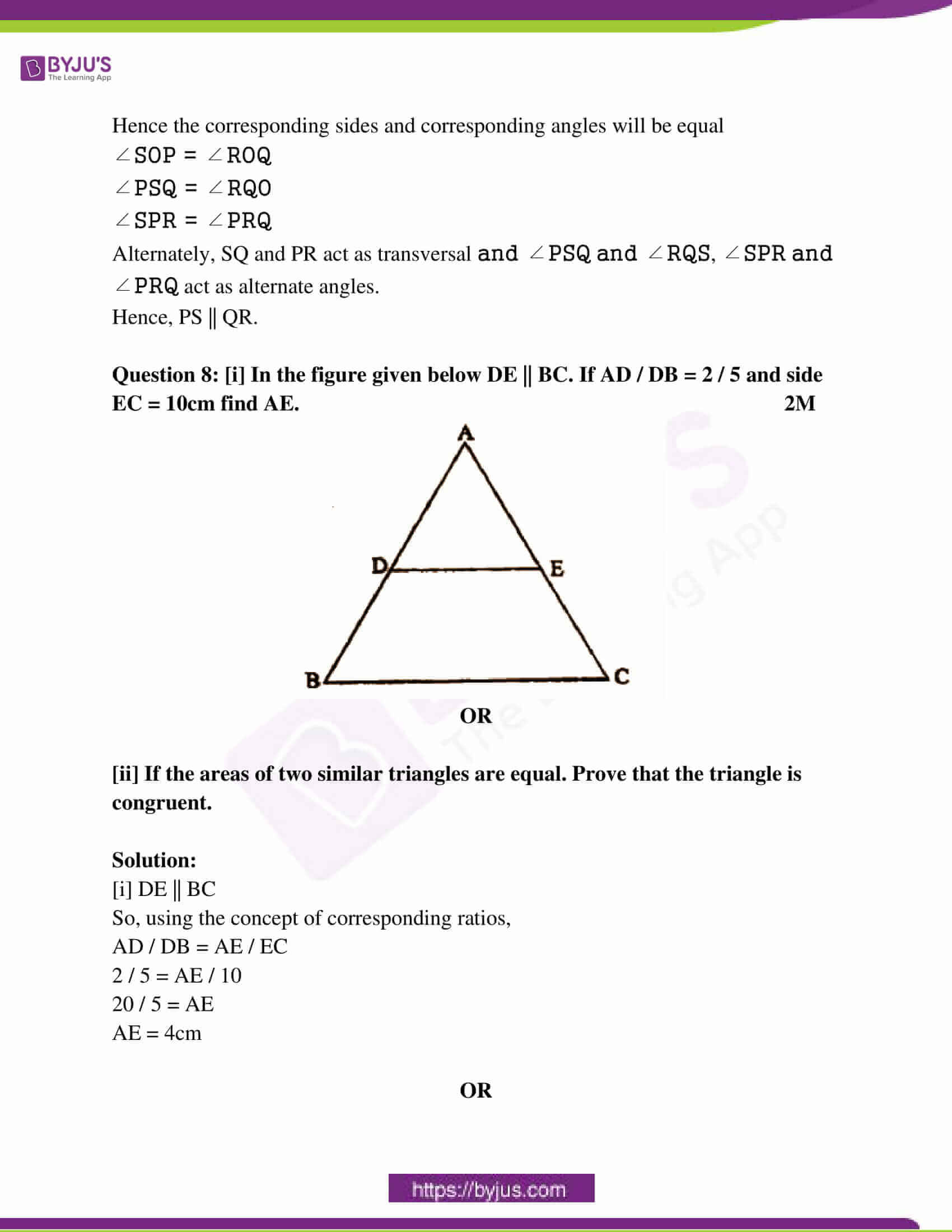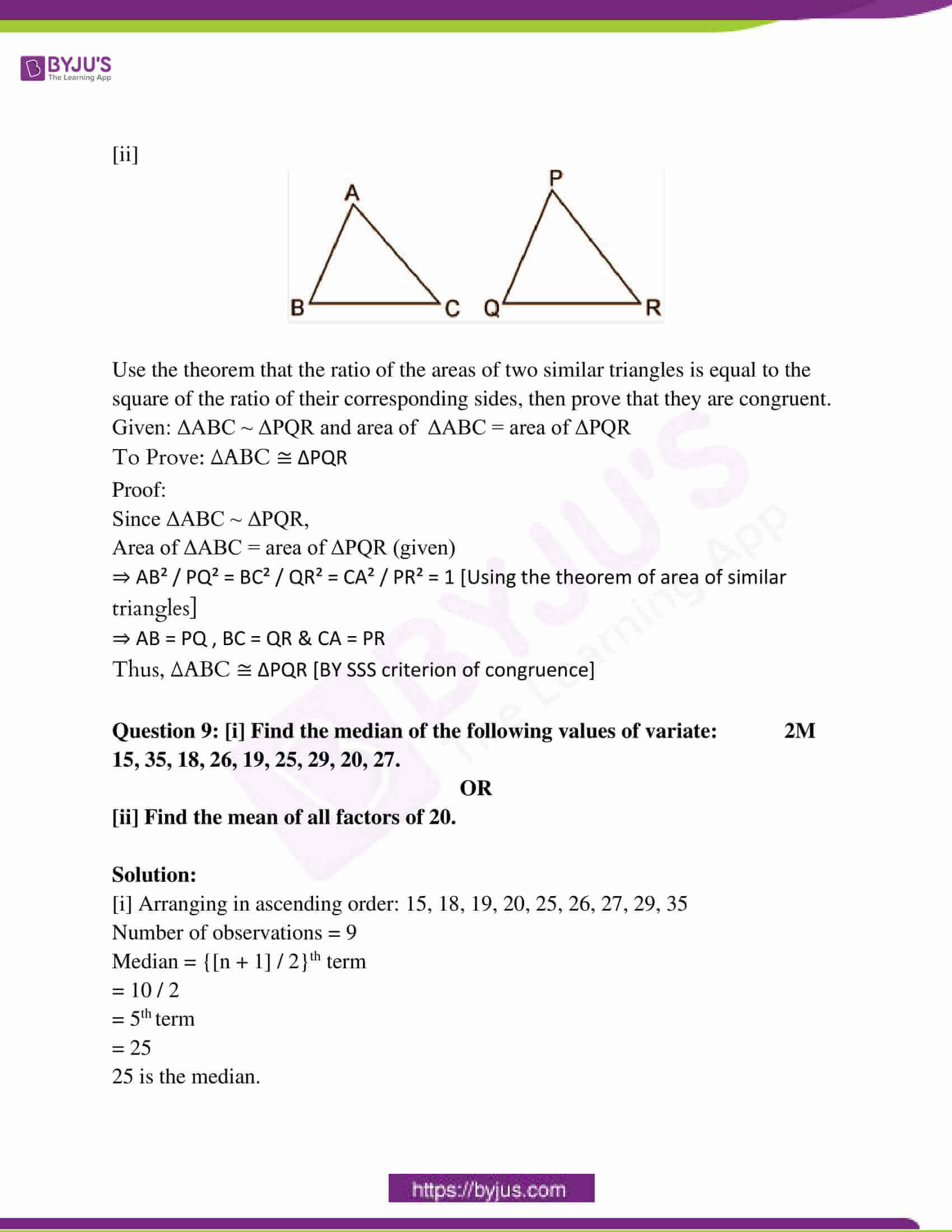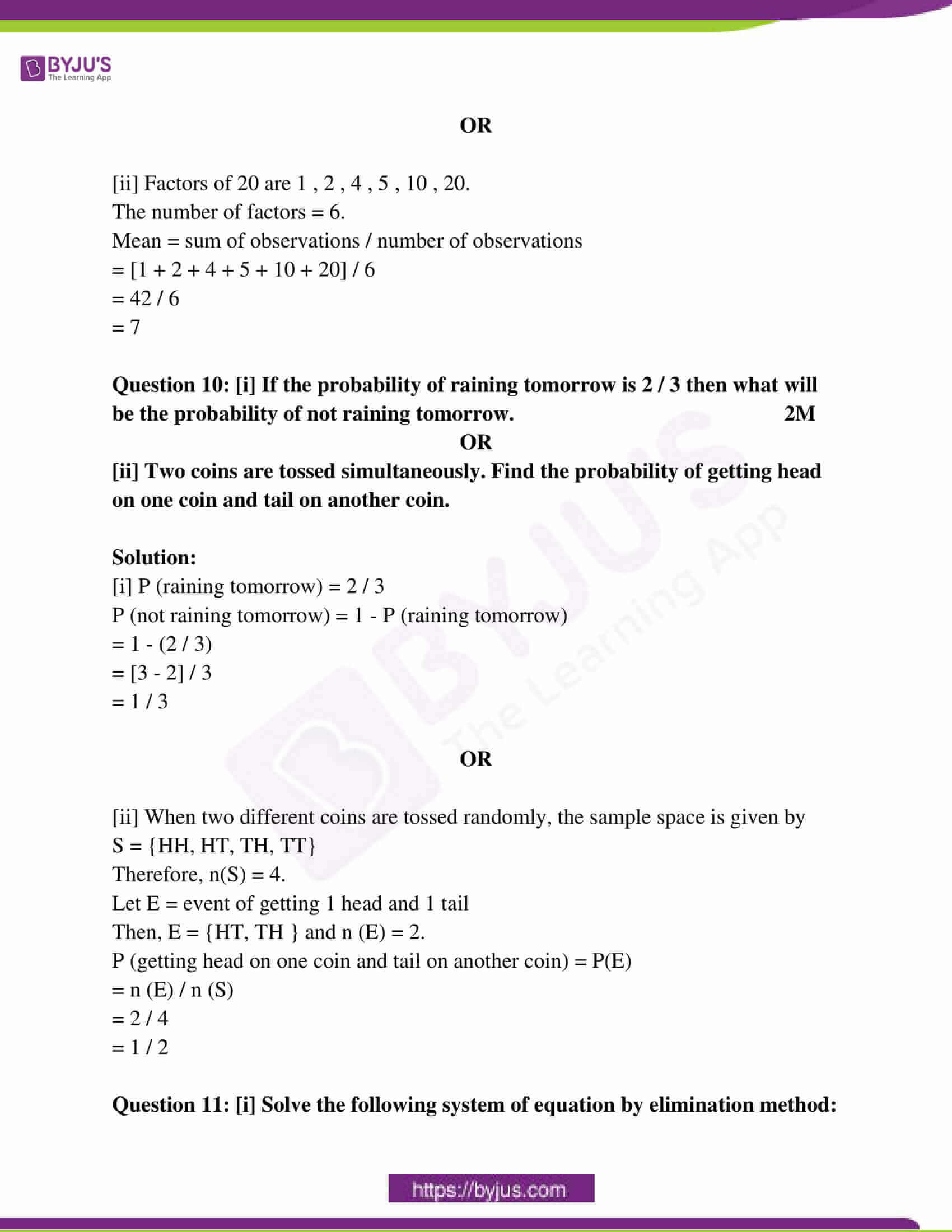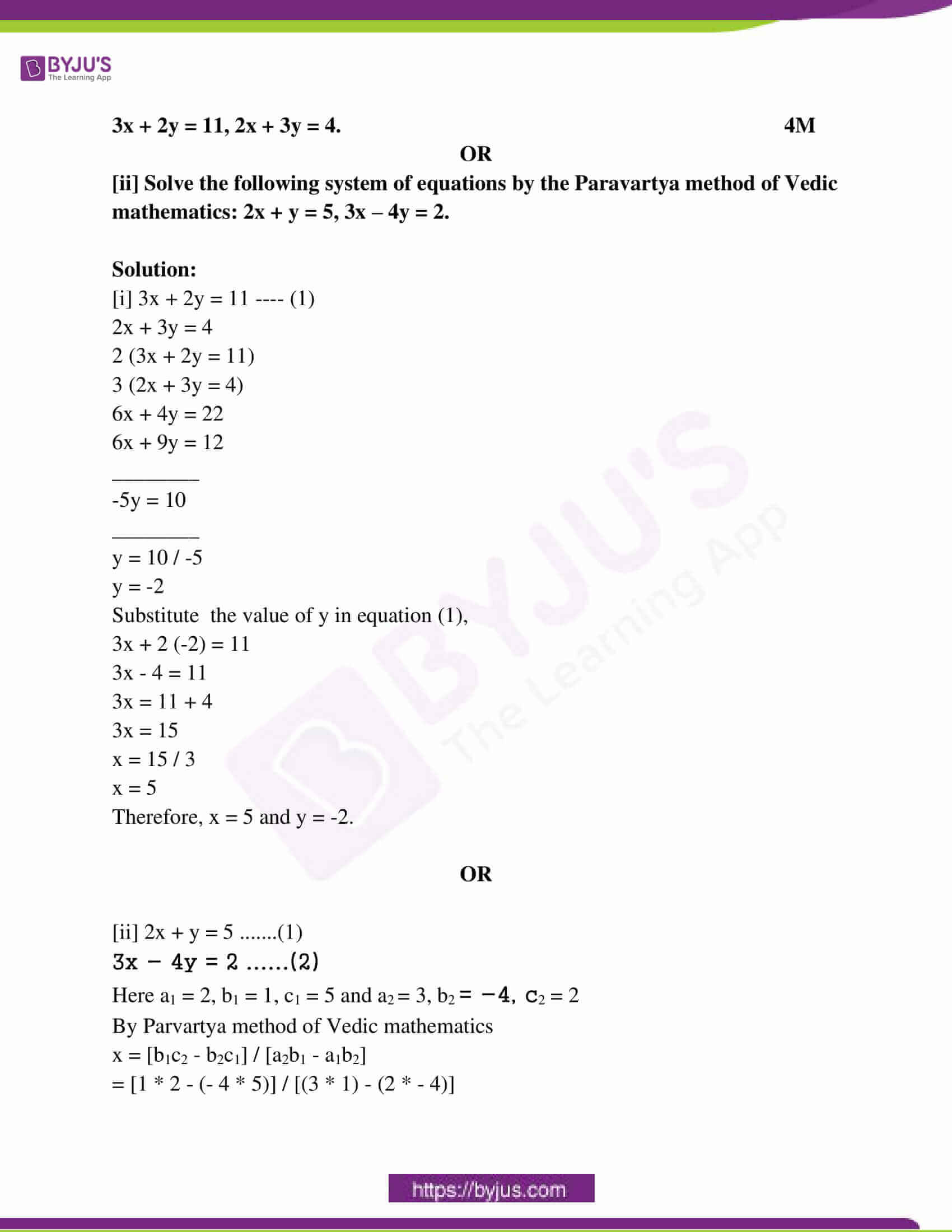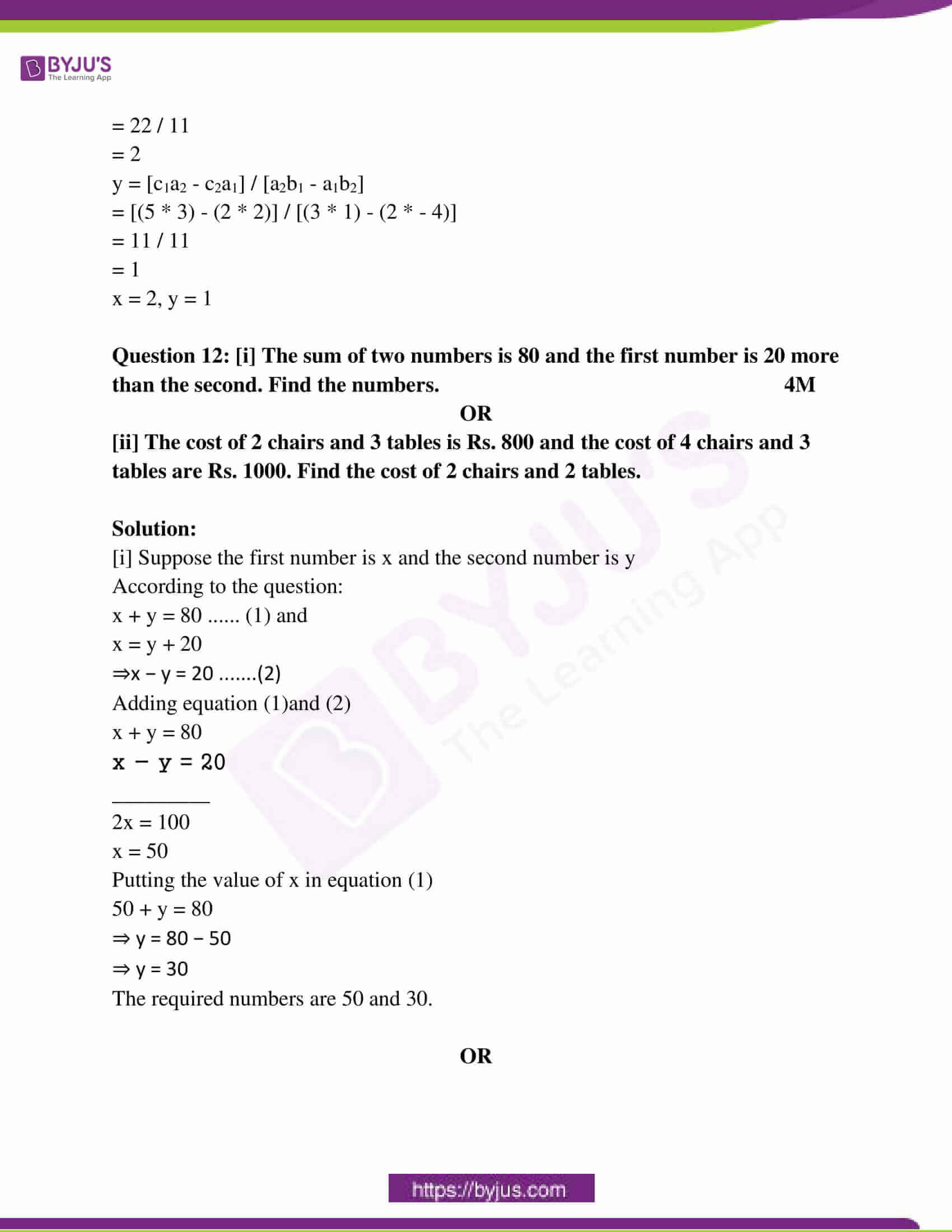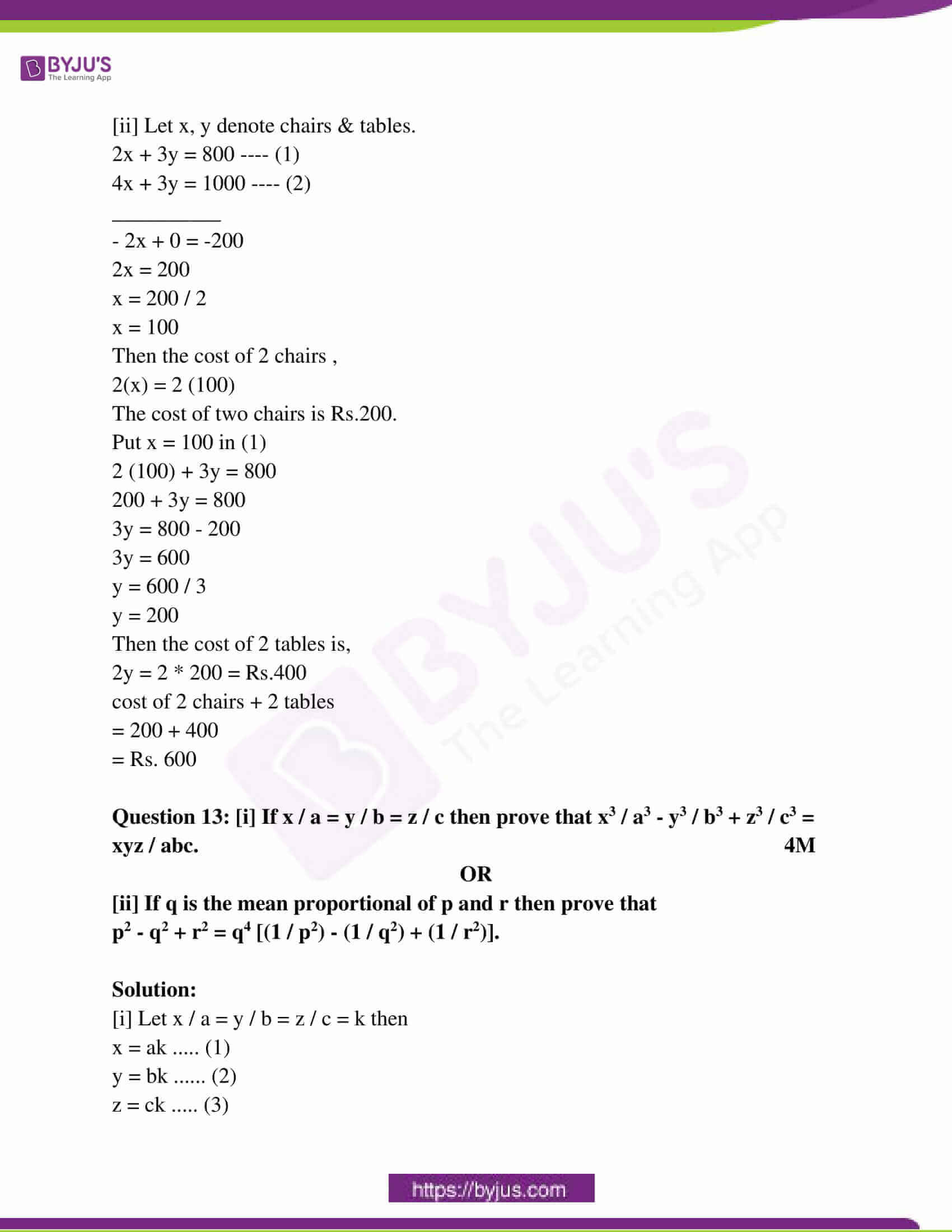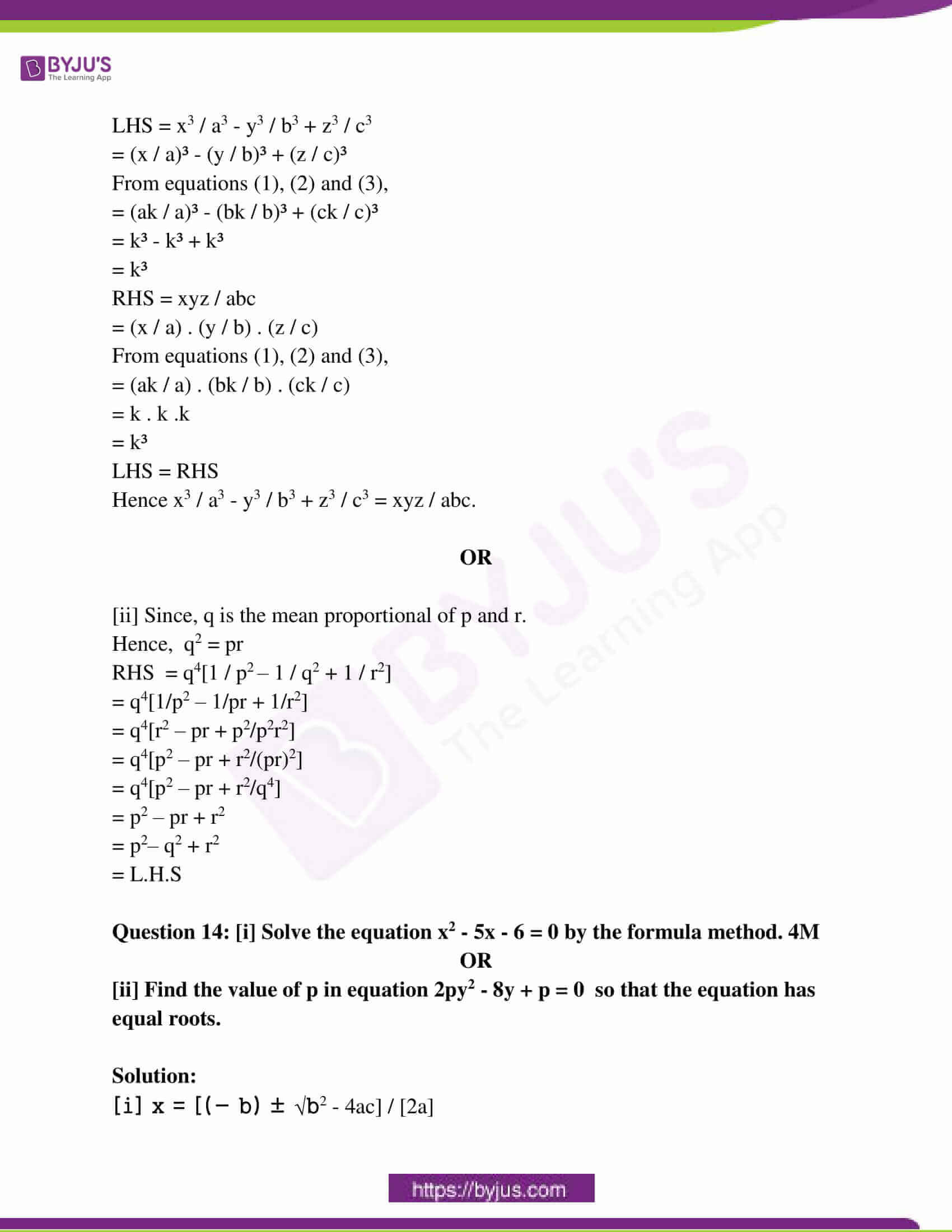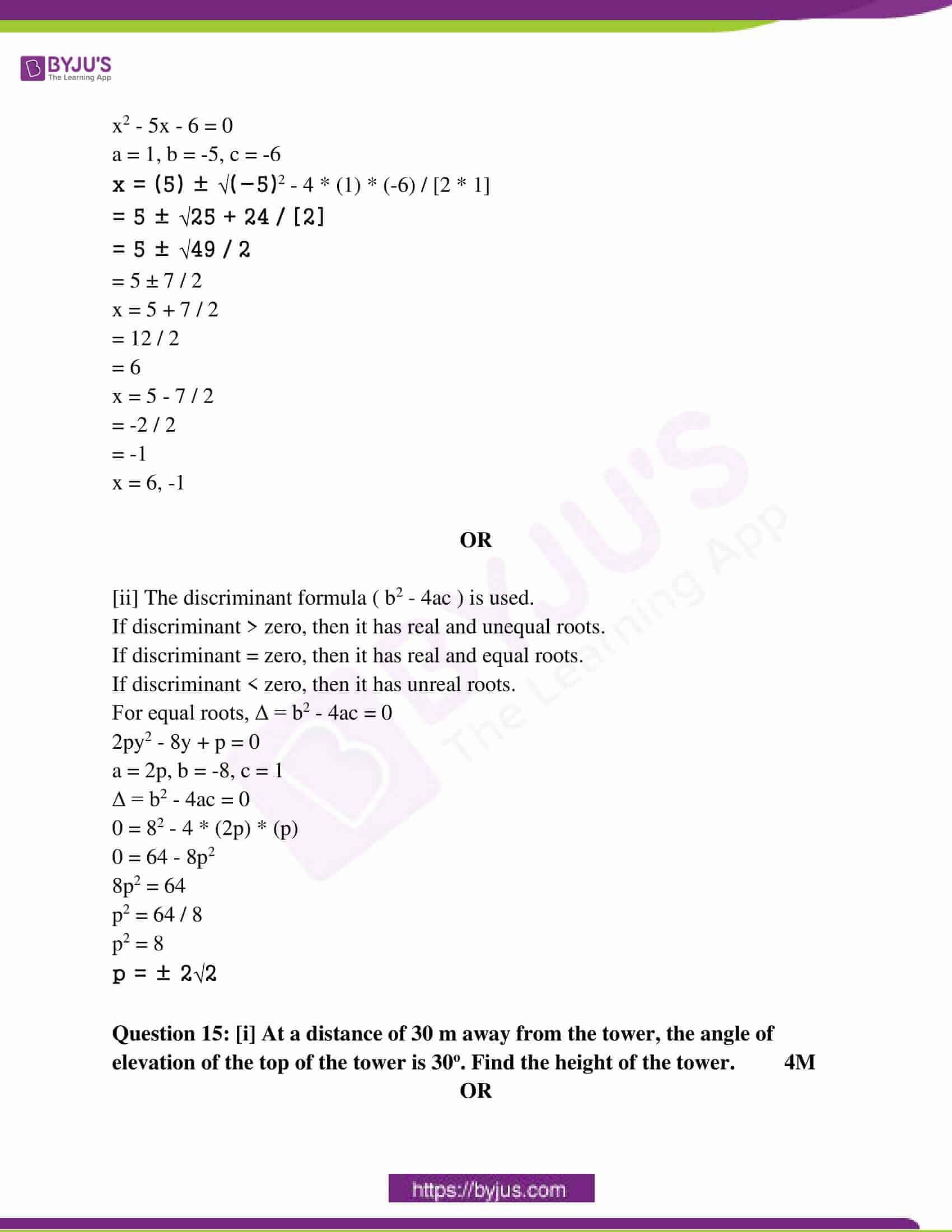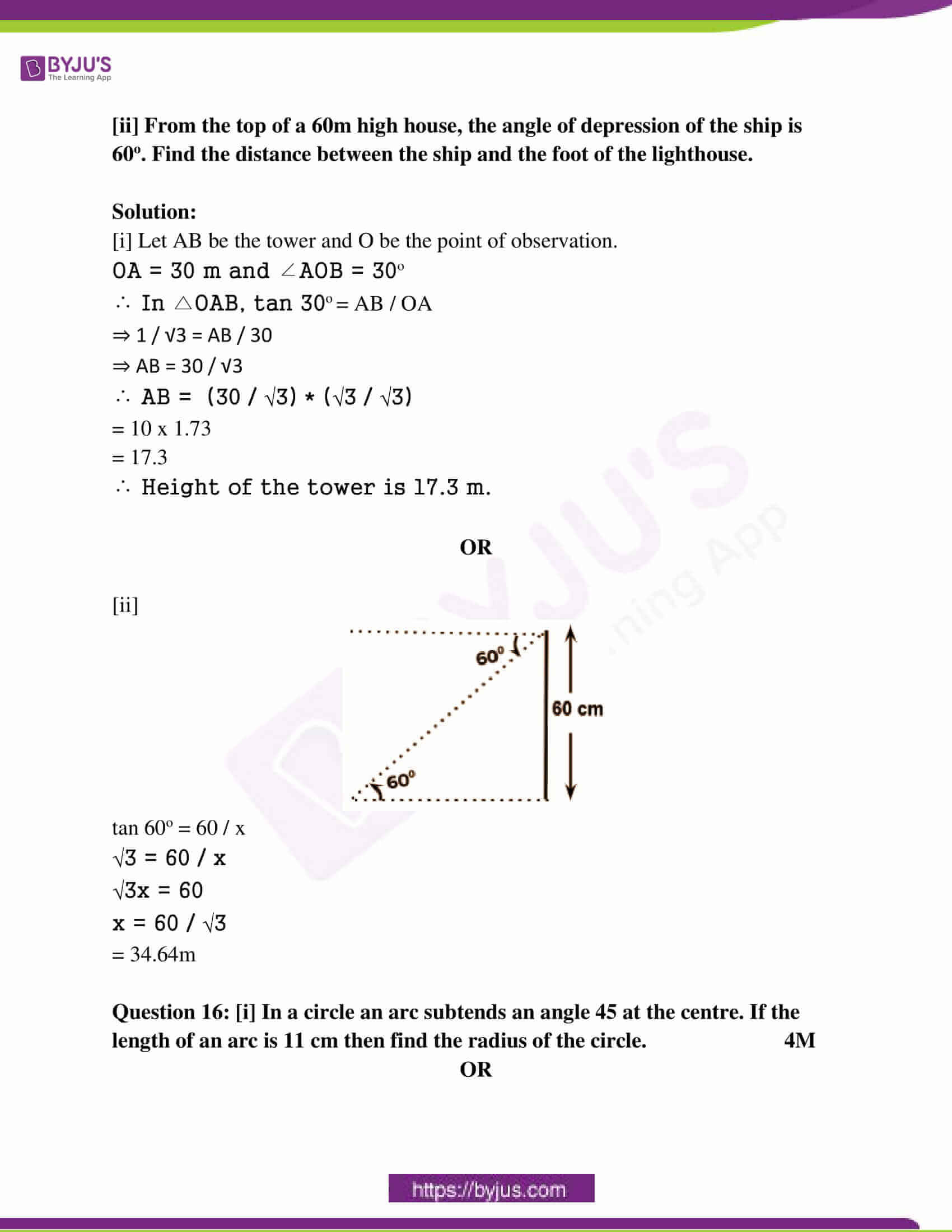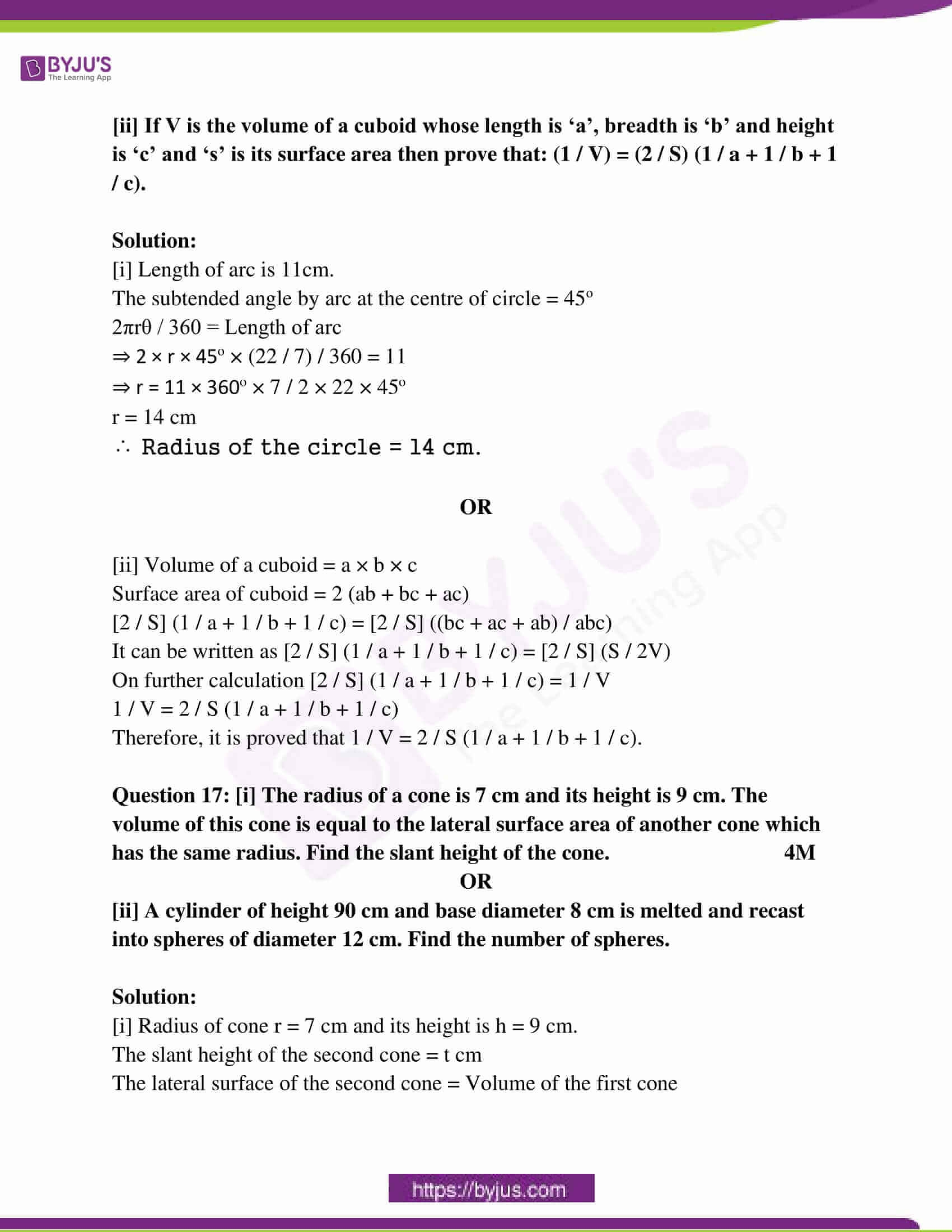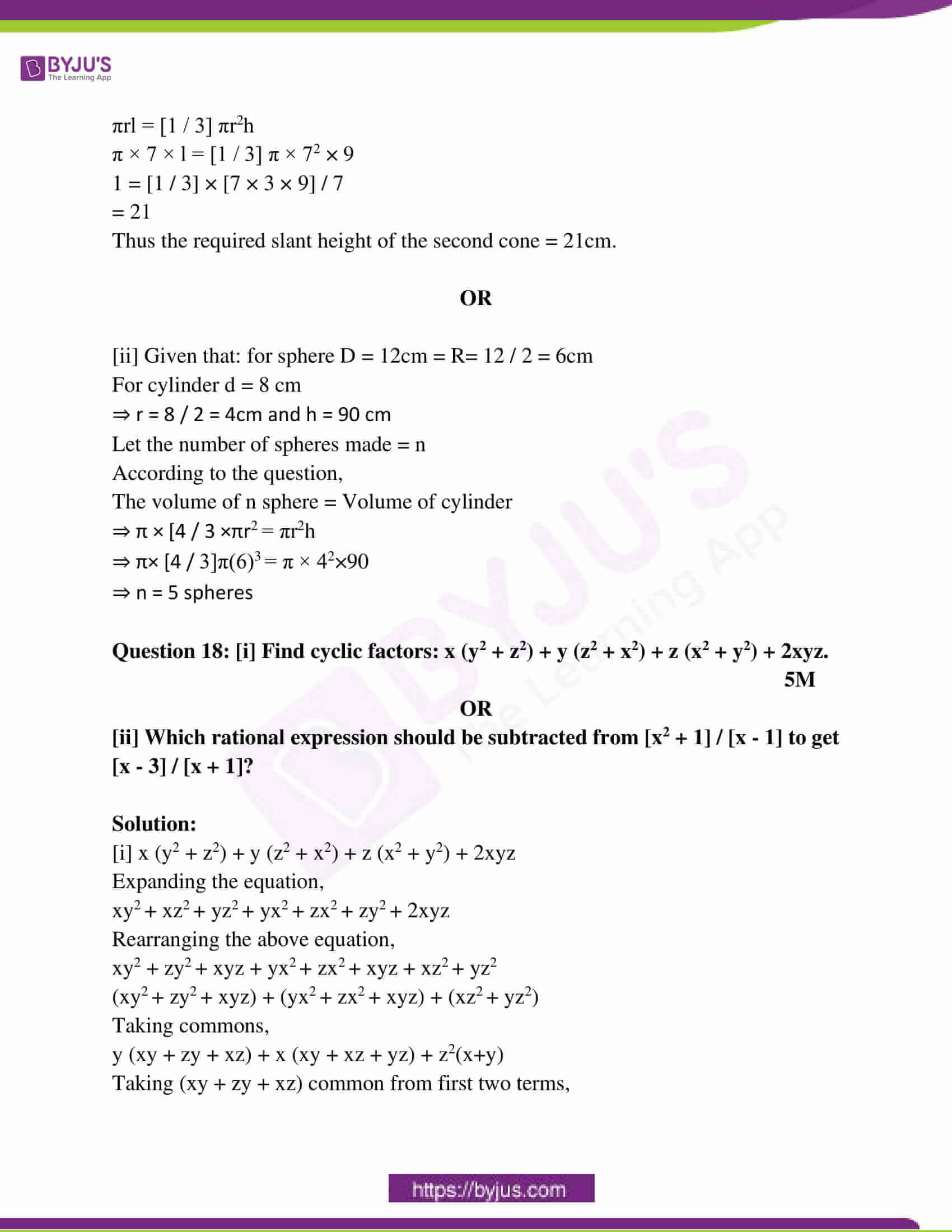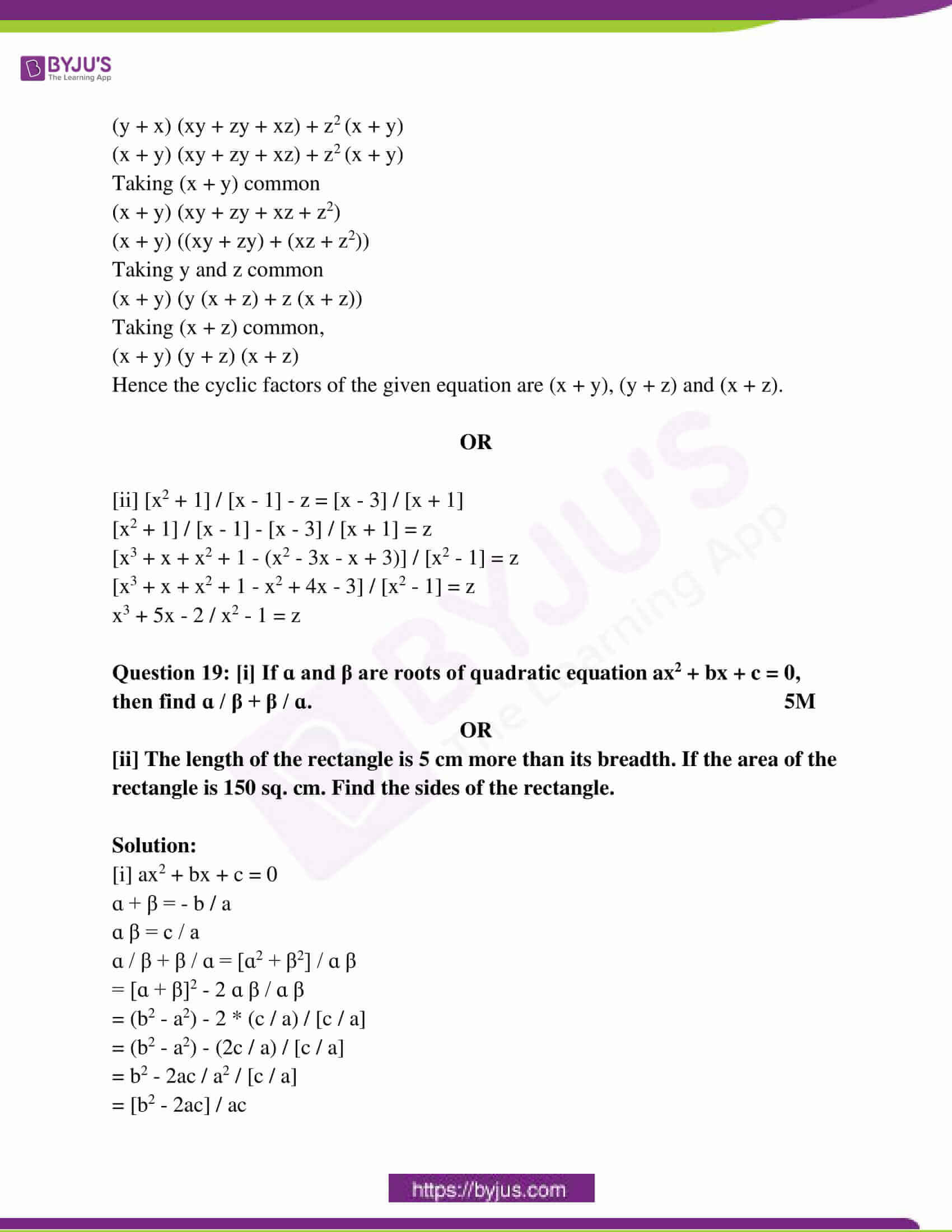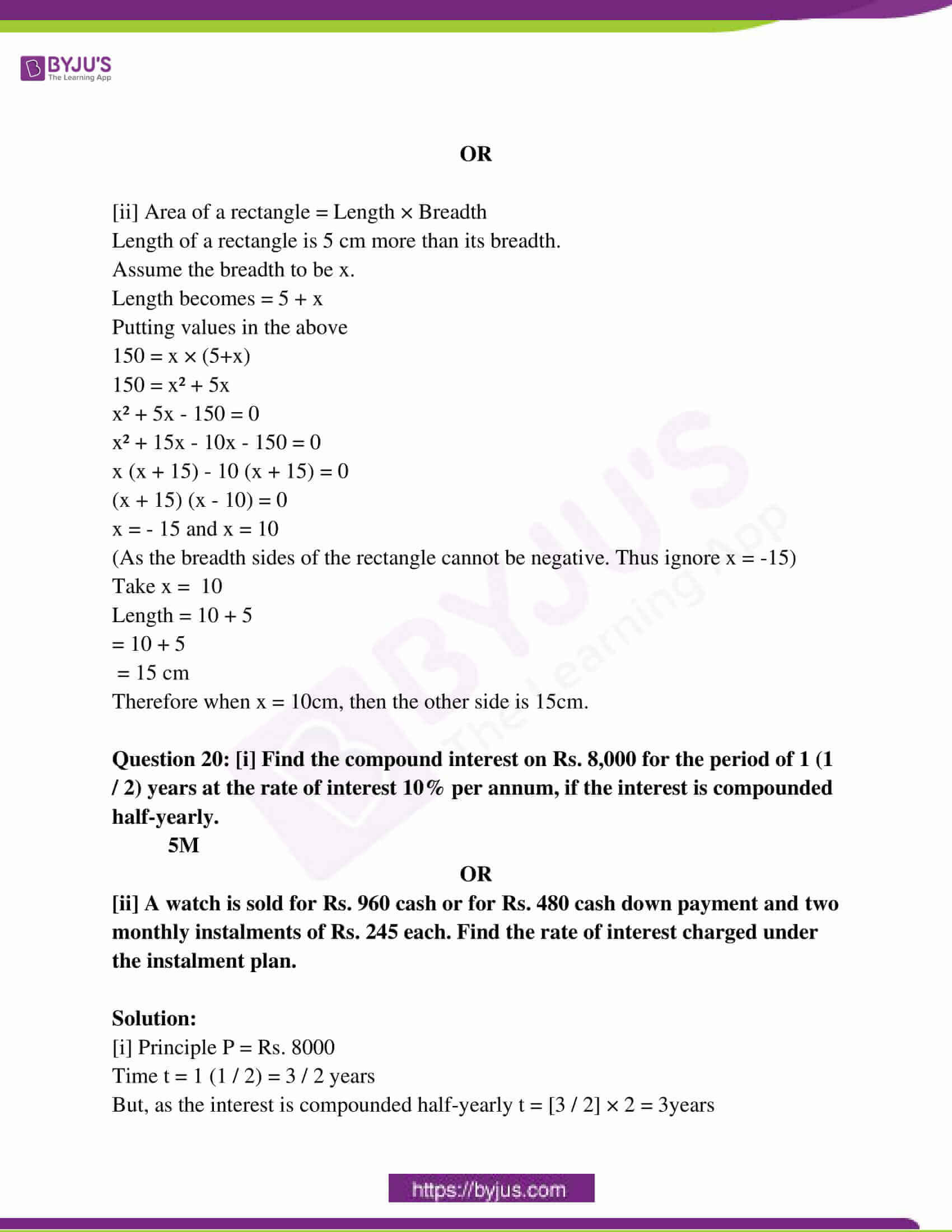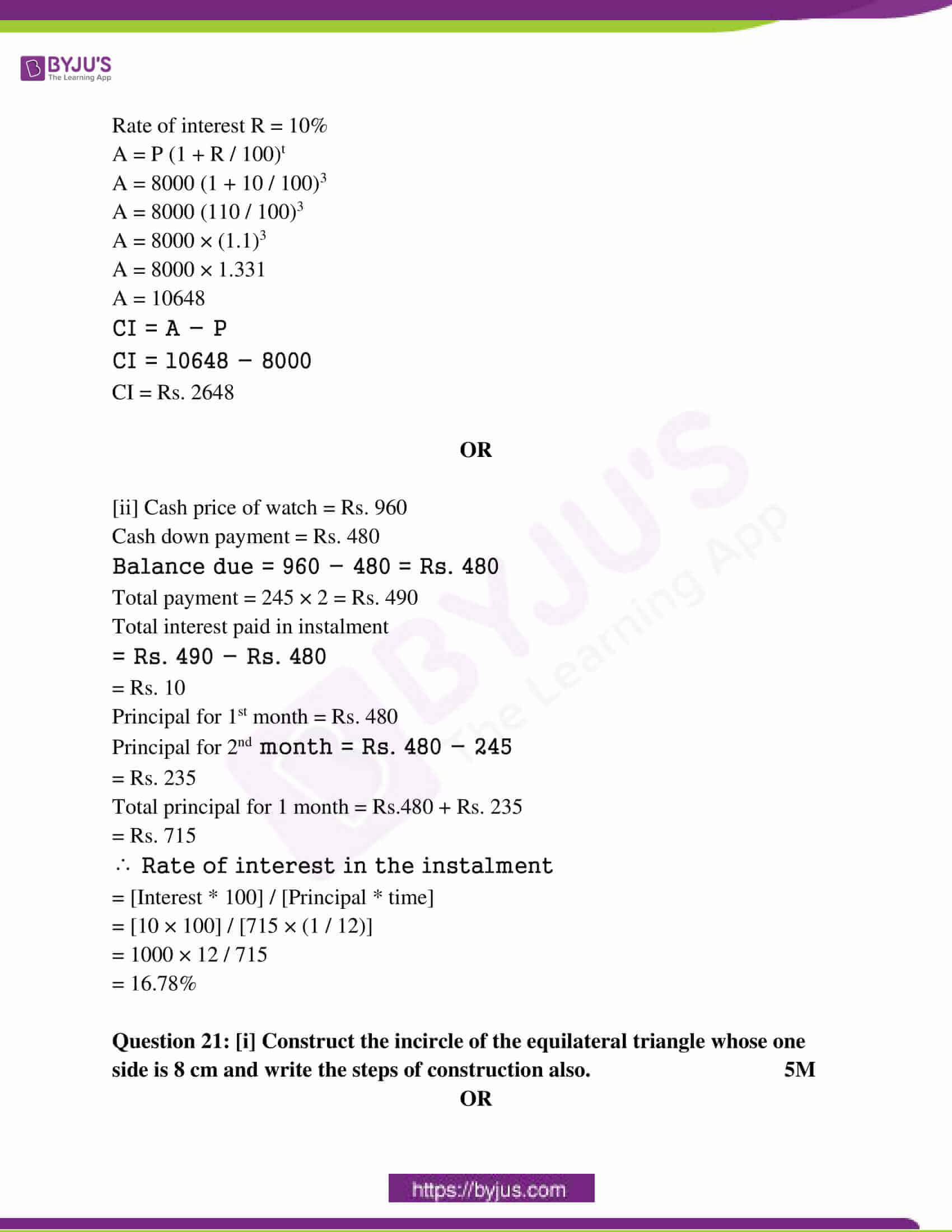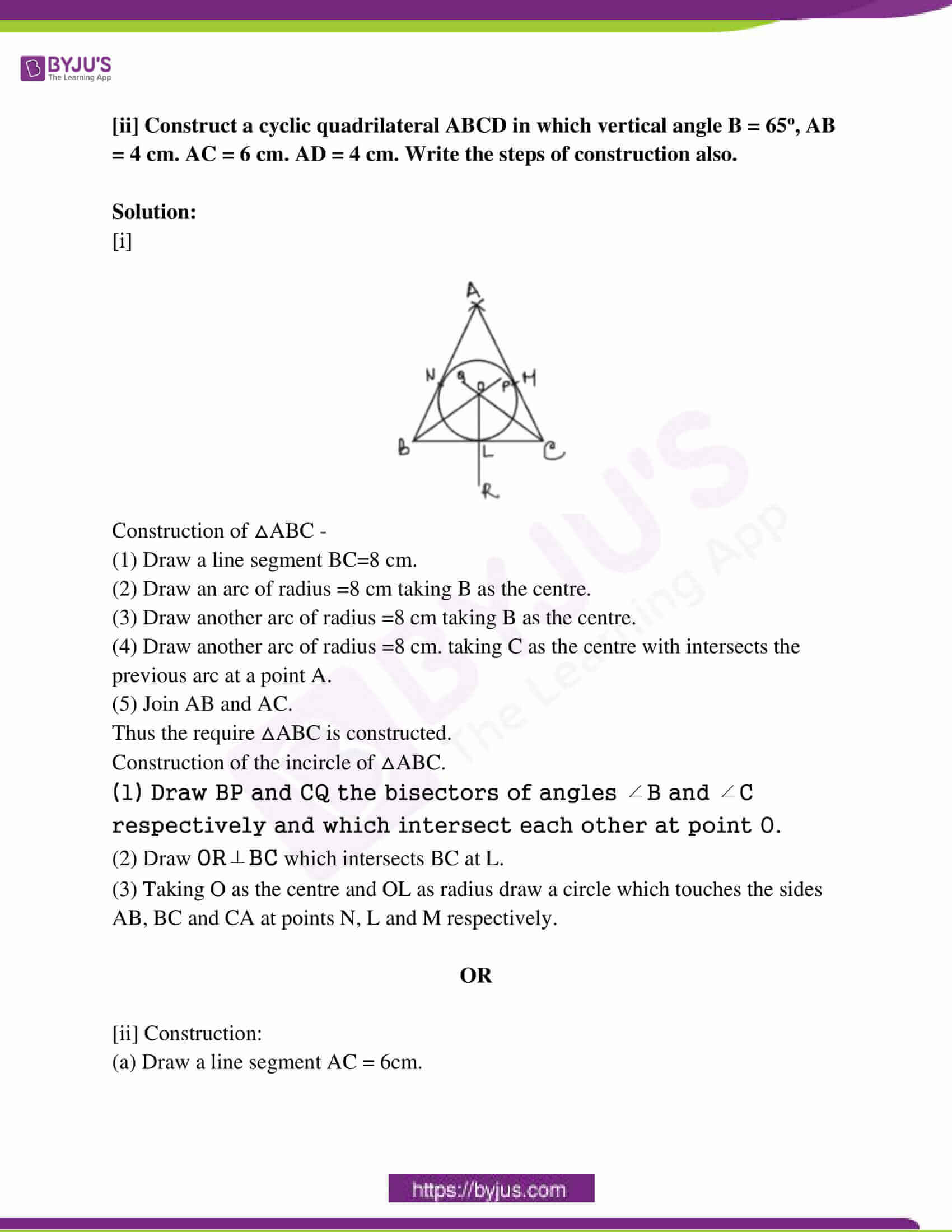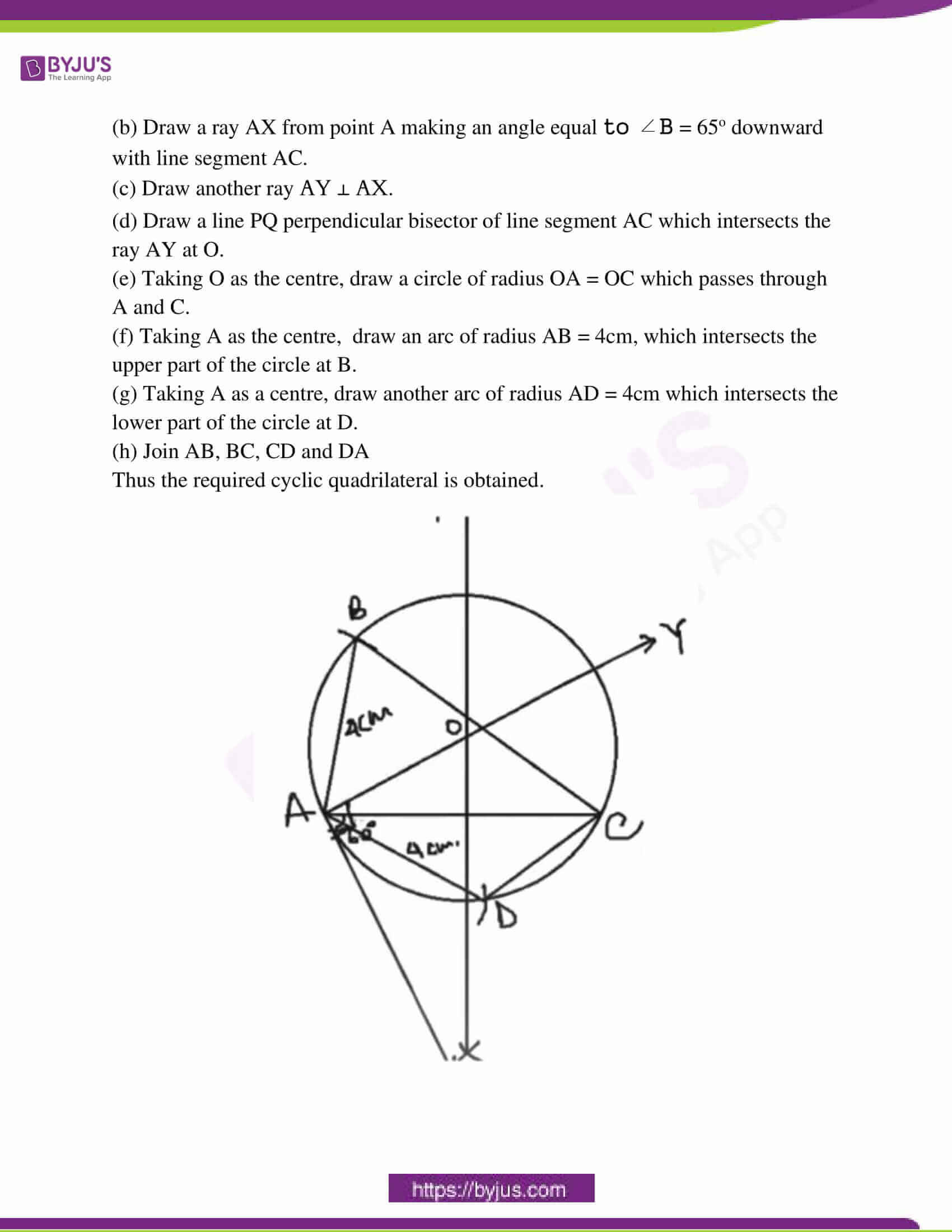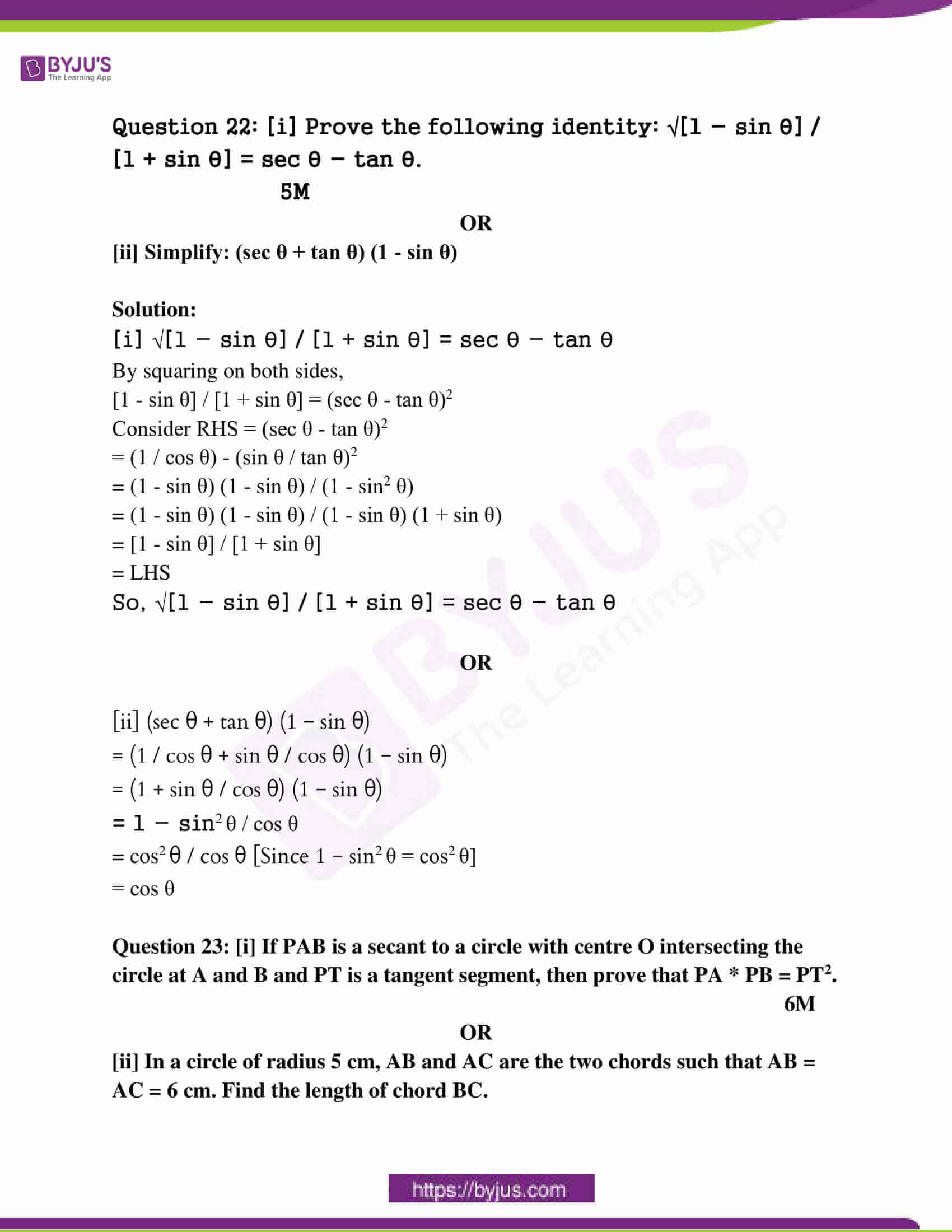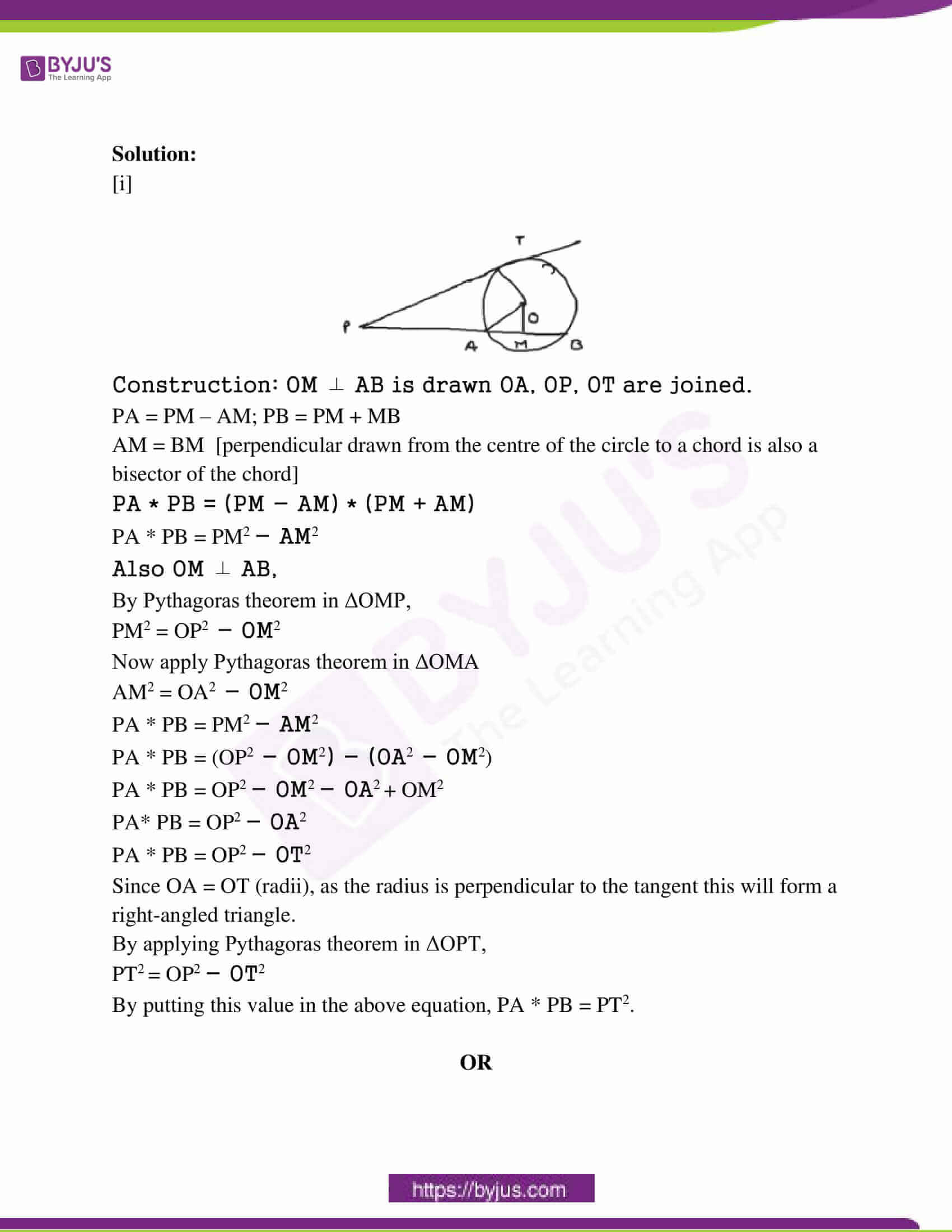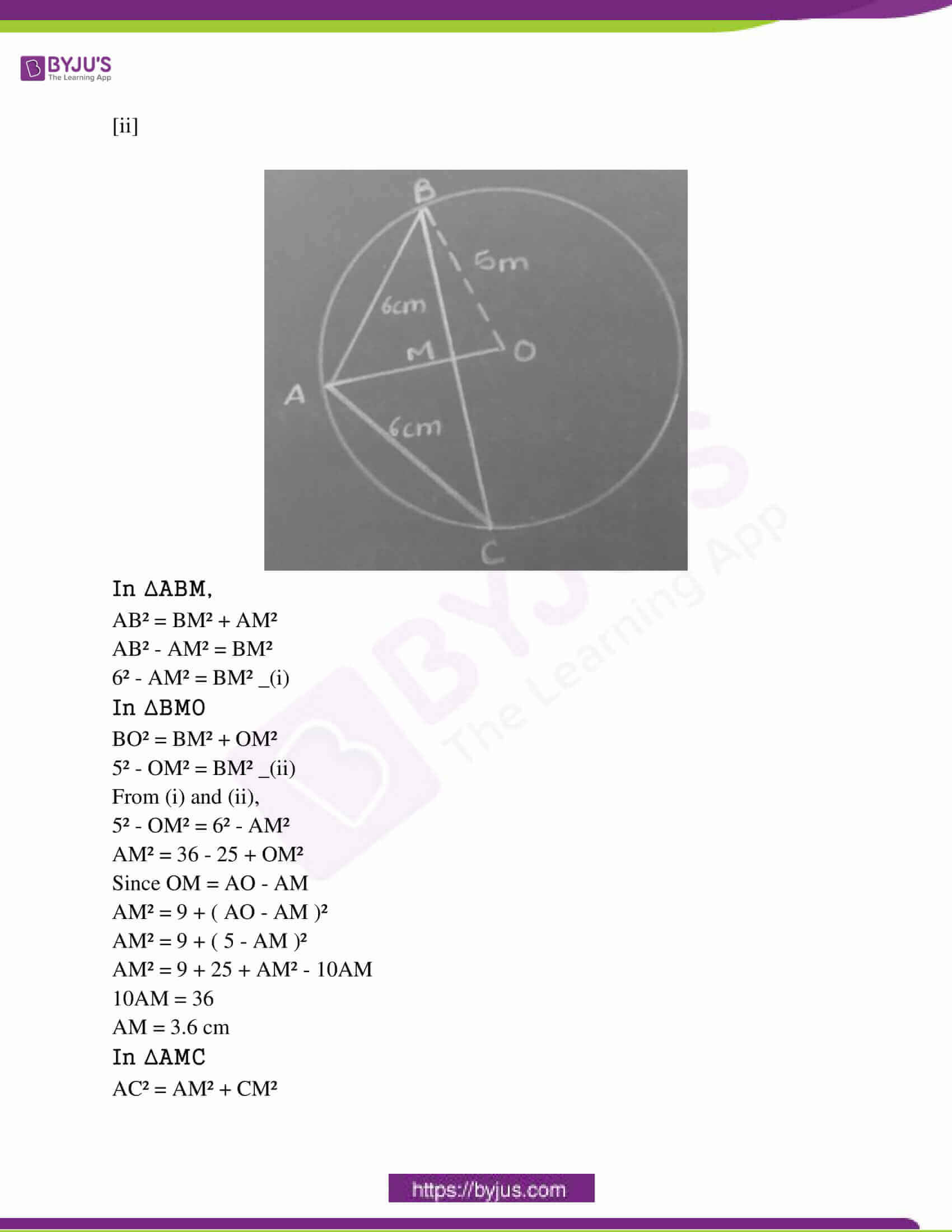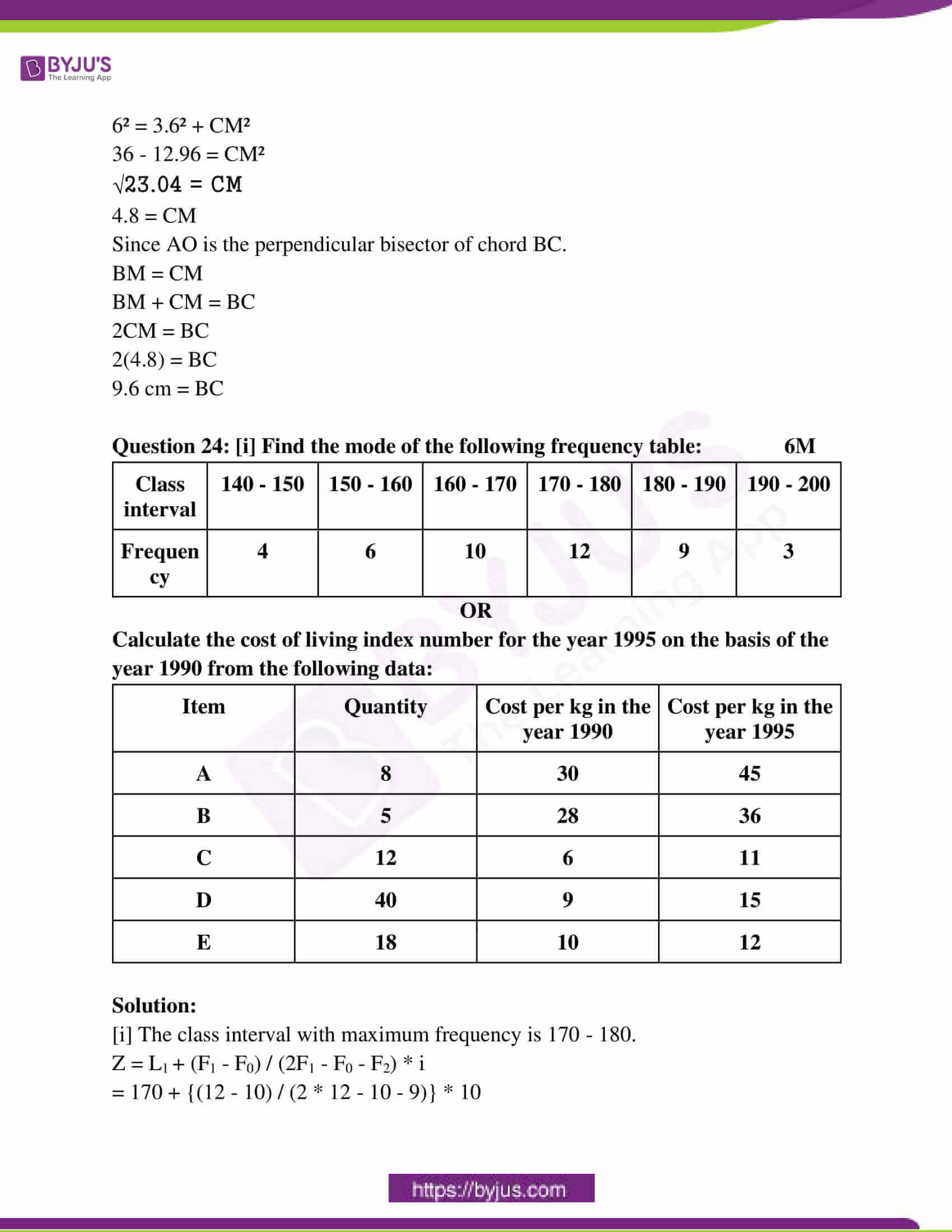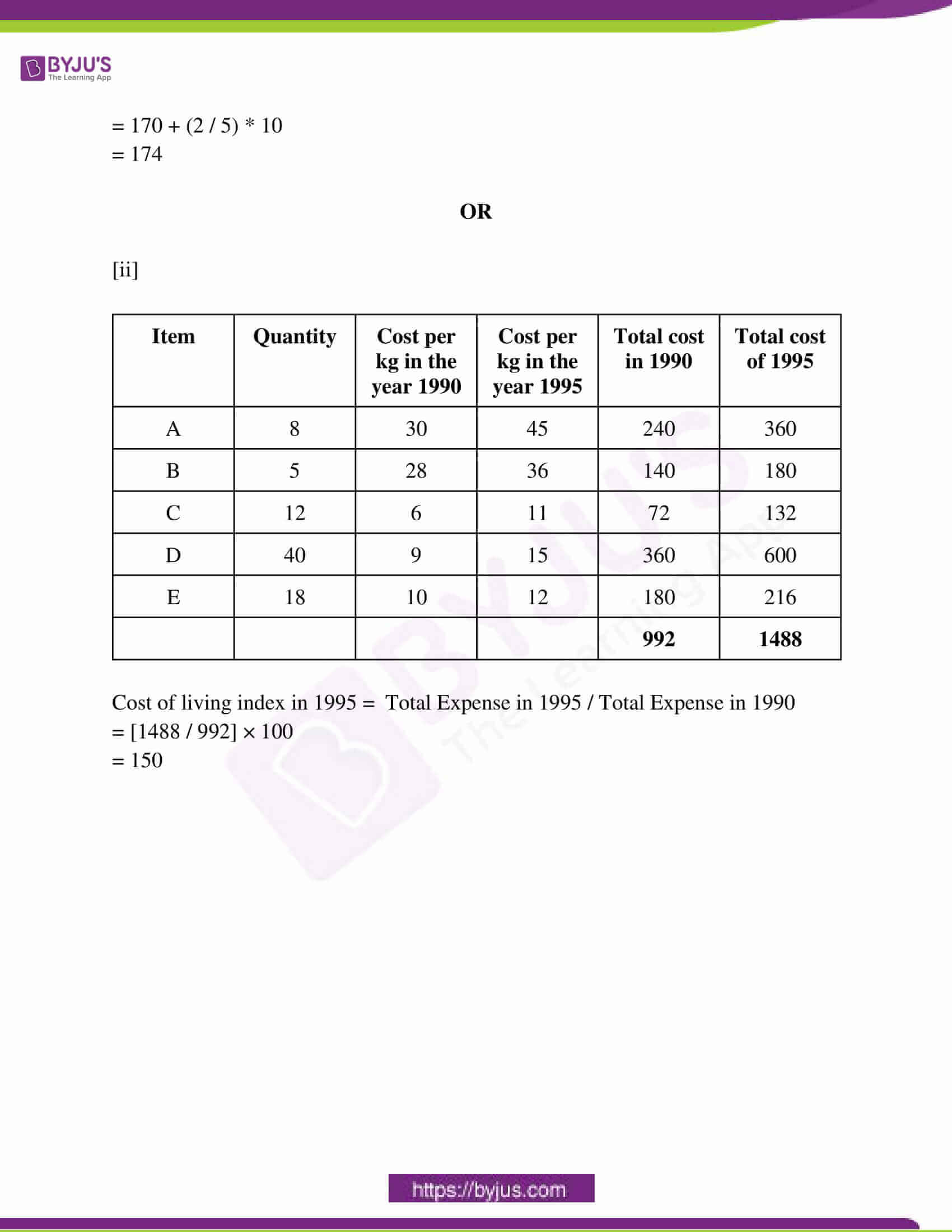Question 1: Choose the correct option and write it in your answer book.

(1 * 5 = 5)

(i) : If a1b2 ≠ a2b1 then the system of equations a1x + b1y + c1 = 0 and a2x + b2y + c2 = 0:

(a) has a unique solution (b) has no solution

(c) has infinitely many solutions (d) has two solutions

(ii) The coordinates of intersection points of lines x + y = 7 and x – y = 3 will be:

(a) (4, 3) (b) (7, 4) (c) (5, 2) (d) (6, 1)

(iii) Zeros of x2 – 2x are

(a) (-2, 0) (b) (2, -2) (c) (0, 2) (d) (1, 2)

(iv) If P = 1 / [x + 1] and Q = x2 – 1 / x2 + 1, then the value of PQ is

(a) x + 1 (b) 1 (c) x – 1 (d) x2 – 1

(v) The third proportional to 8, 12 is

(a) 18 (b) 8 (c) 4 (d) 20

Question 2: Match the following. 1 * 5 = 5

Column A Column B

(1) sin 30o (a) 0

(2) √sec2 θ – 1 (b) cosec θ

(3) sin 55o – cos 35o (c) sin θ

(4) sec θ / tan θ (d) cot θ

(5) √1 – sin2 θ (e) cos 60o

(f) tan θ

1 – e

2 – f

3 – a

4 – b

5 – d

Question 3: Fill in the blanks. 1 * 5 = 5

(i) An equation whose maximum degree of the variable is two is called _____ equation. (quadratic)

(ii) The reduction in the price of the article with time is called _____ (Depreciation)

(iii) If the corresponding angles of two triangles are equal then the triangles are _____ (Similar)

(iv) The solid bounded by two concentric spheres is called _____ (Spherical shell)

(v) The line segment joining the two-point on the circumference of the circle is called _____ (Chord)

Question 4: Write True / False in the following: 1 * 5 = 5

(i) The service tax is an indirect tax. [True]

(ii) The statement of Thales theorem is: “If a line divides any two sides of a triangle in the same ratio, then the line must be parallel to the third side.” [False]

(iii) A circle can be drawn passing through three non-collinear points. [True]

(iv) The length of two tangents drawn from an external point to a circle is unequal. [False]

(v) A line joining the object under consideration with an eye is known as the line of sight. [True]

Question 5: Write the answer is one word/sentence each: 1 * 5 = 5

(i) What is the present rate of education cess?

(ii) What will be the value of x in the figure given below: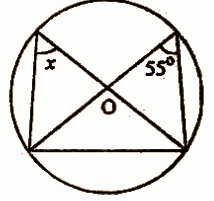(iii): Write the ratio between the volumes of cylinder and cone which have the same radius and height.

(iv): Write the probability of a sure event.

(v): Find the mode of the following observation: 2, 3, 4, 2, 12, 8, 7, 9, 8, 6, 8, 5, 8.

Question 6: [i] Write the statement of Pythagoras theorem. 2M

OR

[ii Check whether 8 cm, 15 cm and 17 cm are the sides of a right-angled triangle.

Solution:

[i] In a right-angled triangle the addition of a square of perpendicular sides equals to the hypotenuse square.

So, H2 = B2 + P2

OR

[ii] On applying Pythagoras on 8, 15, 17,

172 = 82 + 152

289 = 64 + 225

289 = 289

LHS = RHS

Hence, 8 cm, 15 cm and 17 cm are the sides of a right-angled triangle.

Question 7: [i] Are these triangles similar? If yes, then why? If no, then why?

2M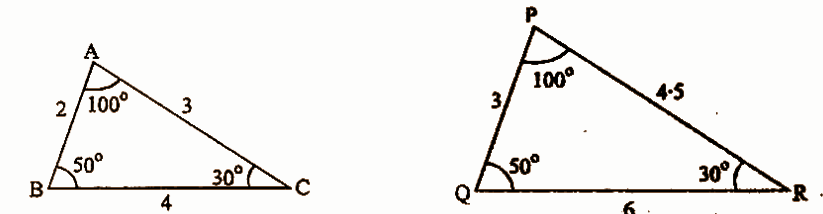OR

[ii] In the figure given below △POS ~ △ROQ, Prove, that PS || QR.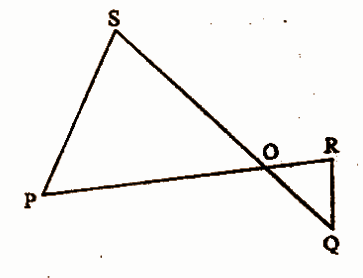Solution:

[i] For the triangles to be similar, the ratio of their corresponding sides and angles is to be taken.

∠A = ∠P

∠B = ∠Q

∠C = ∠R

AB / PQ = BC / QR = AC / PR

2 / 3 = 4 / 6 = 3 / 4.5

So, triangles ABC and PQR are similar.

OR

[ii] △POS ~ △ROQ

Hence the corresponding sides and corresponding angles will be equal

∠SOP = ∠ROQ

∠PSQ = ∠RQO

∠SPR = ∠PRQ

Alternately, SQ and PR act as transversal and ∠PSQ and ∠RQS, ∠SPR and ∠PRQ act as alternate angles.

Hence, PS || QR.

Question 8: [i] In the figure given below DE || BC. If AD / DB = 2 / 5 and side EC = 10cm find AE. 2M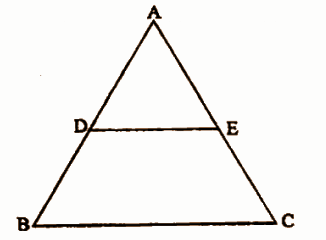OR

[ii] If the areas of two similar triangles are equal. Prove that the triangle is congruent.

Solution:

[i] DE || BC

So, using the concept of corresponding ratios,

AD / DB = AE / EC

2 / 5 = AE / 10

20 / 5 = AE

AE = 4cm

OR

[ii]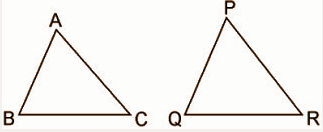Use the theorem that the ratio of the areas of two similar triangles is equal to the square of the ratio of their corresponding sides, then prove that they are congruent.

Given: ΔABC ~ ΔPQR and area of ΔABC = area of ΔPQR

To Prove: ΔABC ≅ ΔPQR

Proof:

Since ΔABC ~ ΔPQR,

Area of ΔABC = area of ΔPQR (given)

⇒ AB² / PQ² = BC² / QR² = CA² / PR² = 1 [Using the theorem of area of similar triangles]

⇒ AB = PQ , BC = QR & CA = PR

Thus, ΔABC ≅ ΔPQR [BY SSS criterion of congruence]

Question 9: [i] Find the median of the following values of variate: 2M

15, 35, 18, 26, 19, 25, 29, 20, 27.

OR

[ii] Find the mean of all factors of 20.

Solution:

[i] Arranging in ascending order: 15, 18, 19, 20, 25, 26, 27, 29, 35

Number of observations = 9

Median = {[n + 1] / 2}th term

= 10 / 2

= 5th term

= 25

25 is the median.

OR

[ii] Factors of 20 are 1 , 2 , 4 , 5 , 10 , 20.

The number of factors = 6.

Mean = sum of observations / number of observations

= [1 + 2 + 4 + 5 + 10 + 20] / 6

= 42 / 6

= 7

Question 10: [i] If the probability of raining tomorrow is 2 / 3 then what will be the probability of not raining tomorrow. 2M

OR

[ii] Two coins are tossed simultaneously. Find the probability of getting head on one coin and tail on another coin.

Solution:

[i] P (raining tomorrow) = 2 / 3

P (not raining tomorrow) = 1 – P (raining tomorrow)

= 1 – (2 / 3)

= [3 – 2] / 3

= 1 / 3

OR

[ii] When two different coins are tossed randomly, the sample space is given by

S = {HH, HT, TH, TT}

Therefore, n(S) = 4.

Let E = event of getting 1 head and 1 tail

Then, E = {HT, TH } and n (E) = 2.

P (getting head on one coin and tail on another coin) = P(E)

= n (E) / n (S)

= 2 / 4

= 1 / 2

Question 11: [i] Solve the following system of equation by elimination method:

3x + 2y = 11, 2x + 3y = 4. 4M

OR

[ii] Solve the following system of equations by the Paravartya method of Vedic mathematics: 2x + y = 5, 3x – 4y = 2.

Solution:

[i] 3x + 2y = 11 —- (1)

2x + 3y = 4

2 (3x + 2y = 11)

3 (2x + 3y = 4)

6x + 4y = 22

6x + 9y = 12

________

-5y = 10

________

y = 10 / -5

y = -2

Substitute the value of y in equation (1),

3x + 2 (-2) = 11

3x – 4 = 11

3x = 11 + 4

3x = 15

x = 15 / 3

x = 5

Therefore, x = 5 and y = -2.

OR

[ii] 2x + y = 5 …….(1)

3x − 4y = 2 ……(2)

Here a1 = 2, b1 = 1, c1 = 5 and a2 = 3, b2 = −4, c2 = 2

By Parvartya method of Vedic mathematics

x = [b1c2 – b2c1] / [a2b1 – a1b2]

= [1 * 2 – (- 4 * 5)] / [(3 * 1) – (2 * – 4)]

= 22 / 11

= 2

y = [c1a2 – c2a1] / [a2b1 – a1b2]

= [(5 * 3) – (2 * 2)] / [(3 * 1) – (2 * – 4)]

= 11 / 11

= 1

x = 2, y = 1

Question 12: [i] The sum of two numbers is 80 and the first number is 20 more than the second. Find the numbers. 4M

OR

[ii] The cost of 2 chairs and 3 tables is Rs. 800 and the cost of 4 chairs and 3 tables are Rs. 1000. Find the cost of 2 chairs and 2 tables.

Solution:

[i] Suppose the first number is x and the second number is y

According to the question:

x + y = 80 …… (1) and

x = y + 20

⇒x − y = 20 …….(2)

Adding equation (1)and (2)

x + y = 80

x − y = 20

_________

2x = 100

x = 50

Putting the value of x in equation (1)

50 + y = 80

⇒ y = 80 − 50

⇒ y = 30

The required numbers are 50 and 30.

OR

[ii] Let x, y denote chairs & tables.

2x + 3y = 800 —- (1)

4x + 3y = 1000 —- (2)

__________

– 2x + 0 = -200

2x = 200

x = 200 / 2

x = 100

Then the cost of 2 chairs ,

2(x) = 2 (100)

The cost of two chairs is Rs.200.

Put x = 100 in (1)

2 (100) + 3y = 800

200 + 3y = 800

3y = 800 – 200

3y = 600

y = 600 / 3

y = 200

Then the cost of 2 tables is,

2y = 2 * 200 = Rs.400

cost of 2 chairs + 2 tables

= 200 + 400

= Rs. 600

Question 13: [i] If x / a = y / b = z / c then prove that x3 / a3 – y3 / b3 + z3 / c3 = xyz / abc. 4M

OR

[ii] If q is the mean proportional of p and r then prove that

p2 – q2 + r2 = q4 [(1 / p2) – (1 / q2) + (1 / r2)].

Solution:

[i] Let x / a = y / b = z / c = k then

x = ak ….. (1)

y = bk …… (2)

z = ck ….. (3)

LHS = x3 / a3 – y3 / b3 + z3 / c3

= (x / a)³ – (y / b)³ + (z / c)³

From equations (1), (2) and (3),

= (ak / a)³ – (bk / b)³ + (ck / c)³

= k³ – k³ + k³

= k³

RHS = xyz / abc

= (x / a) . (y / b) . (z / c)

From equations (1), (2) and (3),

= (ak / a) . (bk / b) . (ck / c)

= k . k .k

= k³

LHS = RHS

Hence x3 / a3 – y3 / b3 + z3 / c3 = xyz / abc.

OR

[ii] Since, q is the mean proportional of p and r.

Hence, q2 = pr

RHS = q4[1 / p2 – 1 / q2 + 1 / r2]

= q4[1/p2 – 1/pr + 1/r2]

= q4[r2 – pr + p2/p2r2]

= q4[p2 – pr + r2/(pr)2]

= q4[p2 – pr + r2/q4]

= p2 – pr + r2

= p2– q2 + r2

= L.H.S

Question 14: [i] Solve the equation x2 – 5x – 6 = 0 by the formula method. 4M

OR

[ii] Find the value of p in equation 2py2 – 8y + p = 0 so that the equation has equal roots.

Solution:

[i] x = [(- b) ± √b2 – 4ac] / [2a]

x2 – 5x – 6 = 0

a = 1, b = -5, c = -6

x = (5) ± √(-5)2 – 4 * (1) * (-6) / [2 * 1]

= 5 ± √25 + 24 / 

= 5 ± √49 / 2

= 5 ± 7 / 2

x = 5 + 7 / 2

= 12 / 2

= 6

x = 5 – 7 / 2

= -2 / 2

= -1

x = 6, -1

OR

[ii] The discriminant formula ( b2 – 4ac ) is used.

If discriminant > zero, then it has real and unequal roots.

If discriminant = zero, then it has real and equal roots.

If discriminant < zero, then it has unreal roots.

For equal roots, Δ = b2 – 4ac = 0

2py2 – 8y + p = 0

a = 2p, b = -8, c = 1

Δ = b2 – 4ac = 0

0 = 82 – 4 * (2p) * (p)

0 = 64 – 8p2

8p2 = 64

p2 = 64 / 8

p2 = 8

p = ± 2√2

Question 15: [i] At a distance of 30 m away from the tower, the angle of elevation of the top of the tower is 30o. Find the height of the tower. 4M

OR

[ii] From the top of a 60m high house, the angle of depression of the ship is 60o. Find the distance between the ship and the foot of the lighthouse.

Solution:

[i] Let AB be the tower and O be the point of observation.

OA = 30 m and ∠AOB = 30o

∴ In △OAB, tan 30o = AB / OA

⇒ 1 / √3 = AB / 30

⇒ AB = 30 / √3

∴ AB = (30 / √3) * (√3 / √3)

= 10 x 1.73

= 17.3

∴ Height of the tower is 17.3 m.

OR

[ii]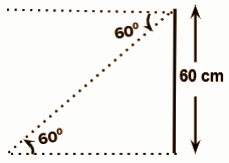tan 60o = 60 / x

√3 = 60 / x

√3x = 60

x = 60 / √3

= 34.64m

Question 16: [i] In a circle an arc subtends an angle 45 at the centre. If the length of an arc is 11 cm then find the radius of the circle. 4M

OR

[ii] If V is the volume of a cuboid whose length is ‘a’, breadth is ‘b’ and height is ‘c’ and ‘s’ is its surface area then prove that: (1 / V) = (2 / S) (1 / a + 1 / b + 1 / c).

Solution:

[i] Length of arc is 11cm.

The subtended angle by arc at the centre of circle = 45o

2πrθ / 360 = Length of arc

⇒ 2 × r × 45o × (22 / 7) / 360 = 11

⇒ r = 11 × 360o × 7 / 2 × 22 × 45o

r = 14 cm

∴ Radius of the circle = 14 cm.

OR

[ii] Volume of a cuboid = a × b × c

Surface area of cuboid = 2 (ab + bc + ac)

[2 / S] (1 / a + 1 / b + 1 / c) = [2 / S] ((bc + ac + ab) / abc)

It can be written as [2 / S] (1 / a + 1 / b + 1 / c) = [2 / S] (S / 2V)

On further calculation [2 / S] (1 / a + 1 / b + 1 / c) = 1 / V

1 / V = 2 / S (1 / a + 1 / b + 1 / c)

Therefore, it is proved that 1 / V = 2 / S (1 / a + 1 / b + 1 / c).

Question 17: [i] The radius of a cone is 7 cm and its height is 9 cm. The volume of this cone is equal to the lateral surface area of another cone which has the same radius. Find the slant height of the cone. 4M

OR

[ii] A cylinder of height 90 cm and base diameter 8 cm is melted and recast into spheres of diameter 12 cm. Find the number of spheres.

Solution:

[i] Radius of cone r = 7 cm and its height is h = 9 cm.

The slant height of the second cone = t cm

The lateral surface of the second cone = Volume of the first cone

πrl = [1 / 3] πr2h

π × 7 × l = [1 / 3] π × 72 × 9

1 = [1 / 3] × [7 × 3 × 9] / 7

​= 21

Thus the required slant height of the second cone = 21cm.

OR

[ii] Given that: for sphere D = 12cm = R= 12 / 2 = 6cm

For cylinder d = 8 cm

⇒ r = 8 / 2 = 4cm and h = 90 cm

Let the number of spheres made = n

According to the question,

The volume of n sphere = Volume of cylinder

⇒ π × [4 / 3 ×πr2 = πr2h

⇒ π× [4 / 3]π(6)3 = π × 42×90

⇒ n = 5 spheres

Question 18: [i] Find cyclic factors: x (y2 + z2) + y (z2 + x2) + z (x2 + y2) + 2xyz.

5M

OR

[ii] Which rational expression should be subtracted from [x2 + 1] / [x – 1] to get [x – 3] / [x + 1]?

Solution:

[i] x (y2 + z2) + y (z2 + x2) + z (x2 + y2) + 2xyz

Expanding the equation,

xy2 + xz2 + yz2 + yx2 + zx2 + zy2 + 2xyz

Rearranging the above equation,

xy2 + zy2 + xyz + yx2 + zx2 + xyz + xz2 + yz2

(xy2 + zy2 + xyz) + (yx2 + zx2 + xyz) + (xz2 + yz2)

Taking commons,

y (xy + zy + xz) + x (xy + xz + yz) + z2(x+y)

Taking (xy + zy + xz) common from first two terms,

(y + x) (xy + zy + xz) + z2 (x + y)

(x + y) (xy + zy + xz) + z2 (x + y)

Taking (x + y) common

(x + y) (xy + zy + xz + z2)

(x + y) ((xy + zy) + (xz + z2))

Taking y and z common

(x + y) (y (x + z) + z (x + z))

Taking (x + z) common,

(x + y) (y + z) (x + z)

Hence the cyclic factors of the given equation are (x + y), (y + z) and (x + z).

OR

[ii] [x2 + 1] / [x – 1] – z = [x – 3] / [x + 1] [x2 + 1] / [x – 1] – [x – 3] / [x + 1] = z

[x3 + x + x2 + 1 – (x2 – 3x – x + 3)] / [x2 – 1] = z

[x3 + x + x2 + 1 – x2 + 4x – 3] / [x2 – 1] = z

x3 + 5x – 2 / x2 – 1 = z

Question 19: [i] If ɑ and β are roots of quadratic equation ax2 + bx + c = 0, then find ɑ / β + β / ɑ. 5M

OR

[ii] The length of the rectangle is 5 cm more than its breadth. If the area of the rectangle is 150 sq. cm. Find the sides of the rectangle.

Solution:

[i] ax2 + bx + c = 0

ɑ + β = – b / a

ɑ β = c / a

ɑ / β + β / ɑ = [ɑ2 + β2] / ɑ β

= [ɑ + β]2 – 2 ɑ β / ɑ β

= (b2 – a2) – 2 * (c / a) / [c / a]

= (b2 – a2) – (2c / a) / [c / a]

= b2 – 2ac / a2 / [c / a]

= [b2 – 2ac] / ac

OR

[ii] Area of a rectangle = Length × Breadth

Length of a rectangle is 5 cm more than its breadth.

Assume the breadth to be x.

Length becomes = 5 + x

Putting values in the above

150 = x × (5+x)

150 = x² + 5x

x² + 5x – 150 = 0

x² + 15x – 10x – 150 = 0

x (x + 15) – 10 (x + 15) = 0

(x + 15) (x – 10) = 0

x = – 15 and x = 10

(As the breadth sides of the rectangle cannot be negative. Thus ignore x = -15)

Take x = 10

Length = 10 + 5

= 10 + 5

= 15 cm

Therefore when x = 10cm, then the other side is 15cm.

Question 20: [i] Find the compound interest on Rs. 8,000 for the period of 1 (1 / 2) years at the rate of interest 10% per annum, if the interest is compounded half-yearly. 5M

OR

[ii] A watch is sold for Rs. 960 cash or for Rs. 480 cash down payment and two monthly instalments of Rs. 245 each. Find the rate of interest charged under the instalment plan.

Solution:

[i] Principle P = Rs. 8000

Time t = 1 (1 / 2) = 3 / 2 years

But, as the interest is compounded half-yearly t = [3 / 2] × 2 = 3years

Rate of interest R = 10%

A = P (1 + R / 100)t

A = 8000 (1 + 10 / 100)3

A = 8000 (110 / 100)3

A = 8000 × (1.1)3

A = 8000 × 1.331

A = 10648

CI = A − P

CI = 10648 − 8000

CI = Rs. 2648

OR

[ii] Cash price of watch = Rs. 960

Cash down payment = Rs. 480

Balance due = 960 − 480 = Rs. 480

Total payment = 245 × 2 = Rs. 490

Total interest paid in instalment

= Rs. 490 − Rs. 480

= Rs. 10

Principal for 1st month = Rs. 480

Principal for 2nd month = Rs. 480 − 245

= Rs. 235

Total principal for 1 month = Rs.480 + Rs. 235

= Rs. 715

∴ Rate of interest in the instalment

= [Interest * 100] / [Principal * time]

= [10 × 100] / [715 × (1 / 12)]

= 1000 × 12 / 715

= 16.78%

Question 21: [i] Construct the incircle of the equilateral triangle whose one side is 8 cm and write the steps of construction also. 5M

OR

[ii] Construct a cyclic quadrilateral ABCD in which vertical angle B = 65o, AB = 4 cm. AC = 6 cm. AD = 4 cm. Write the steps of construction also.

Solution:

[i]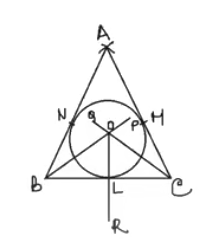Construction of △ABC –

(1) Draw a line segment BC=8 cm.

(2) Draw an arc of radius =8 cm taking B as the centre.

(3) Draw another arc of radius =8 cm taking B as the centre.

(4) Draw another arc of radius =8 cm. taking C as the centre with intersects the previous arc at a point A.

(5) Join AB and AC.

Thus the require △ABC is constructed.

Construction of the incircle of △ABC.

(1) Draw BP and CQ the bisectors of angles ∠B and ∠C respectively and which intersect each other at point O.

(2) Draw OR⊥BC which intersects BC at L.

(3) Taking O as the centre and OL as radius draw a circle which touches the sides AB, BC and CA at points N, L and M respectively.

OR

[ii] Construction:

(a) Draw a line segment AC = 6cm.

(b) Draw a ray AX from point A making an angle equal to ∠B = 65o downward with line segment AC.

(c) Draw another ray AY ⟂ AX.

(d) Draw a line PQ perpendicular bisector of line segment AC which intersects the ray AY at O.

(e) Taking O as the centre, draw a circle of radius OA = OC which passes through A and C.

(f) Taking A as the centre, draw an arc of radius AB = 4cm, which intersects the upper part of the circle at B.

(g) Taking A as a centre, draw another arc of radius AD = 4cm which intersects the lower part of the circle at D.

(h) Join AB, BC, CD and DA

Thus the required cyclic quadrilateral is obtained.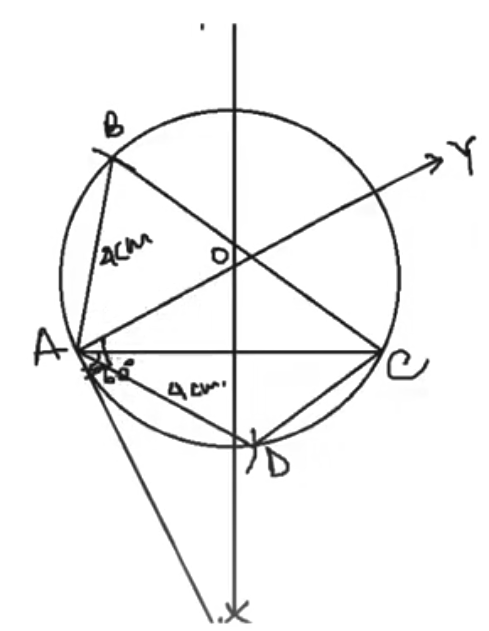Question 22: [i] Prove the following identity: √[1 – sin θ] / [1 + sin θ] = sec θ – tan θ. 5M

OR

[ii] Simplify: (sec θ + tan θ) (1 – sin θ)

Solution:

[i] √[1 – sin θ] / [1 + sin θ] = sec θ – tan θ

By squaring on both sides,

[1 – sin θ] / [1 + sin θ] = (sec θ – tan θ)2

Consider RHS = (sec θ – tan θ)2

= (1 / cos θ) – (sin θ / tan θ)2

= (1 – sin θ) (1 – sin θ) / (1 – sin2 θ)

= (1 – sin θ) (1 – sin θ) / (1 – sin θ) (1 + sin θ)

= [1 – sin θ] / [1 + sin θ]

= LHS

So, √[1 – sin θ] / [1 + sin θ] = sec θ – tan θ

OR

[ii] (sec θ + tan θ) (1 − sin θ)

= (1 / cos θ + sin θ / cos θ) (1 − sin θ)

= (1 + sin θ / cos θ) (1 − sin θ)

= 1 − sin2 θ / cos θ

= cos2 θ / cos θ [Since 1 − sin2 θ = cos2 θ]

= cos θ

Question 23: [i] If PAB is a secant to a circle with centre O intersecting the circle at A and B and PT is a tangent segment, then prove that PA * PB = PT2.

6M

OR

[ii] In a circle of radius 5 cm, AB and AC are the two chords such that AB = AC = 6 cm. Find the length of chord BC.

Solution:

[i]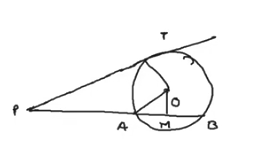Construction: OM ⊥ AB is drawn OA, OP, OT are joined.

PA = PM – AM; PB = PM + MB

AM = BM [perpendicular drawn from the centre of the circle to a chord is also a bisector of the chord]

PA * PB = (PM − AM) * (PM + AM)

PA * PB = PM2 − AM2

Also OM ⊥ AB,

By Pythagoras theorem in ΔOMP,

PM2 = OP2 − OM2

Now apply Pythagoras theorem in ΔOMA

AM2 = OA2 − OM2

PA * PB = PM2 − AM2

PA * PB = (OP2 − OM2) − (OA2 − OM2)

PA * PB = OP2 − OM2 − OA2 + OM2

PA* PB = OP2 − OA2

PA * PB = OP2 − OT2

Since OA = OT (radii), as the radius is perpendicular to the tangent this will form a right-angled triangle.

By applying Pythagoras theorem in ΔOPT,

PT2 = OP2 − OT2

By putting this value in the above equation, PA * PB = PT2.

OR

[ii]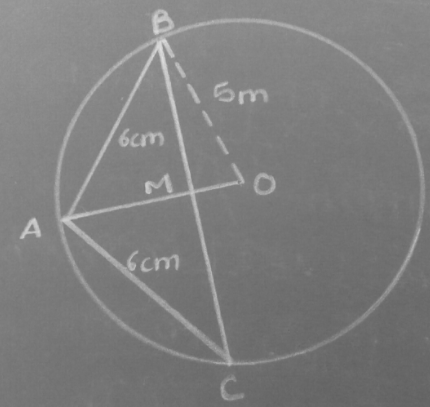In ∆ABM,

AB² = BM² + AM²

AB² – AM² = BM²

6² – AM² = BM² _(i)

In ∆BMO

BO² = BM² + OM²

5² – OM² = BM² _(ii)

From (i) and (ii),

5² – OM² = 6² – AM²

AM² = 36 – 25 + OM²

Since OM = AO – AM

AM² = 9 + ( AO – AM )²

AM² = 9 + ( 5 – AM )²

AM² = 9 + 25 + AM² – 10AM

10AM = 36

AM = 3.6 cm

In ∆AMC

AC² = AM² + CM²

6² = 3.6² + CM²

36 – 12.96 = CM²

√23.04 = CM

4.8 = CM

Since AO is the perpendicular bisector of chord BC.

BM = CM

BM + CM = BC

2CM = BC

2(4.8) = BC

9.6 cm = BC

Question 24: [i] Find the mode of the following frequency table: 6M

 Class interval 140 – 150 150 – 160 160 – 170 170 – 180 180 – 190 190 – 200 Frequency 4 6 10 12 9 3

OR

Calculate the cost of living index number for the year 1995 on the basis of the year 1990 from the following data:

 Item Quantity Cost per kg in the year 1990 Cost per kg in the year 1995 A 8 30 45 B 5 28 36 C 12 6 11 D 40 9 15 E 18 10 12

Solution:

[i] The class interval with maximum frequency is 170 – 180.

Z = L1 + (F1 – F0) / (2F1 – F0 – F2) * i

= 170 + {(12 – 10) / (2 * 12 – 10 – 9)} * 10

= 170 + (2 / 5) * 10

= 174

OR

[ii]
 Item Quantity Cost per kg in the year 1990 Cost per kg in the year 1995 Total cost in 1990 Total cost of 1995 A 8 30 45 240 360 B 5 28 36 140 180 C 12 6 11 72 132 D 40 9 15 360 600 E 18 10 12 180 216 992 1488

Cost of living index in 1995 = Total Expense in 1995 / Total Expense in 1990

= [1488 / 992] × 100

= 150